# Bayesian Missing Data Imputation#

import random

import arviz as az
import matplotlib.cm as cm
import matplotlib.pyplot as plt
import numpy as np
import pandas as pd
import pymc as pm
import scipy.optimize

from matplotlib.lines import Line2D
from pymc.sampling.jax import sample_blackjax_nuts, sample_numpyro_nuts
from scipy.stats import multivariate_normal

/Users/nathanielforde/opt/miniconda3/envs/missing_data_clean/lib/python3.11/site-packages/pymc/sampling/jax.py:39: UserWarning: This module is experimental.
warnings.warn("This module is experimental.")


## Bayesian Imputation and Degrees of Missing-ness#

The analysis of data with missing values is a gateway into the study of causal inference.

One of the key features of any analysis plagued by missing data is the assumption which governs the nature of the missing-ness i.e. what is the reason for gaps in our data? Can we ignore them? Should we worry about why? In this notebook we’ll see an example of how to handle missing data using maximum likelihood estimation and bayesian imputation techniques. This will open up questions about the assumptions governing inference in the presence of missing data, and inference in counterfactual cases.

We will make the discussion concrete by considering an example analysis of an employee satisfaction survey and how different work conditions contribute to the responses and non-responses we see in the data.

%config InlineBackend.figure_format = 'retina'  # high resolution figures
az.style.use("arviz-darkgrid")
rng = np.random.default_rng(42)


## Missing Data Taxonomy#

Rubin’s famous taxonomy breaks out the question into a choice of three fundamental options:

• Missing Completely at Random (MCAR)

• Missing at Random (MAR)

• Missing Not at Random (MNAR)

Each of these paradigms can be reduced to explicit definition in terms of the conditional probability regarding the pattern of missing data. The first pattern is the least concerning. The (MCAR) assumption states that the data are missing in a manner that is unrelated to both the observed and unobserved parts of the realised data. It is missing due to the haphazard circumstance of the world $$\phi$$.

$P(M =1 | Y_{obs}, Y_{miss}, \phi) = P(M =1 | \phi)$

whereas the second pattern (MAR) allows that the reasons for missingness can be function of the observed data and circumstances of the world. Some times this is called a case of ignorable missingness because estimation can proceed in good faith on the basis of the observed data. There may be a loss of precision, but the inference should be sound.

$P(M =1 | Y_{obs}, Y_{miss}, \phi) = P(M =1 | Y_{obs}, \phi)$

The most nefarious sort of missing data is when the missingness is a function of something outside the observed data, and the equation cannot be reduced further. Efforts at imputation and estimation more generally may become more difficulty in this final case because of the risk of confounding. This is a case of non-ignorable missing-ness.

$P(M =1 | Y_{obs}, Y_{miss}, \phi)$

These assumptions are made before any analysis begins. They are inherently unverifiable. Your analysis will stand or fall depending on how plausible each assumption is in the context you seek to apply them. For example, an another type missing data results from systematic censoring as discussed in Bayesian regression with truncated or censored data. In such cases the reason for censoring governs the missing-ness pattern.

## Employee Satisfaction Surveys#

We’ll follow the presentation of Craig Enders’ Applied Missing Data Analysis Enders K  and work with employee satisifaction data set. The data set comprises of a few composite measures reporting employee working conditions and satisfactions. Of particular note are empowerment (empower), work satisfaction (worksat) and two composite survey scores recording the employees leadership climate (climate), and the relationship quality with their supervisor lmx.

The key question is what assumptions governs our patterns of missing data.

try:
except FileNotFoundError:

employee team turnover male empower lmx worksat climate cohesion
0 1 1 0.0 1 32.0 11.0 3.0 18.0 3.5
1 2 1 1.0 1 NaN 13.0 4.0 18.0 3.5
2 3 1 1.0 1 30.0 9.0 4.0 18.0 3.5
3 4 1 1.0 1 29.0 8.0 3.0 18.0 3.5
4 5 1 1.0 0 26.0 7.0 4.0 18.0 3.5
# Percentage Missing
df_employee[["worksat", "empower", "lmx"]].isna().sum() / len(df_employee)

worksat    0.047619
empower    0.161905
lmx        0.041270
dtype: float64

# Patterns of missing Data
df_employee[["worksat", "empower", "lmx"]].isnull().drop_duplicates().reset_index(drop=True)

worksat empower lmx
0 False False False
1 False True False
2 True True False
3 False False True
4 True False False
fig, ax = plt.subplots(figsize=(20, 7))
ax.hist(df_employee["empower"], bins=30, ec="black", color="cyan", label="Empowerment")
ax.hist(df_employee["lmx"], bins=30, ec="black", color="yellow", label="LMX")
ax.hist(df_employee["worksat"], bins=30, ec="black", color="green", label="Work Satisfaction")
ax.set_title("Employee Satisfaction Survey Results", fontsize=20)
ax.legend();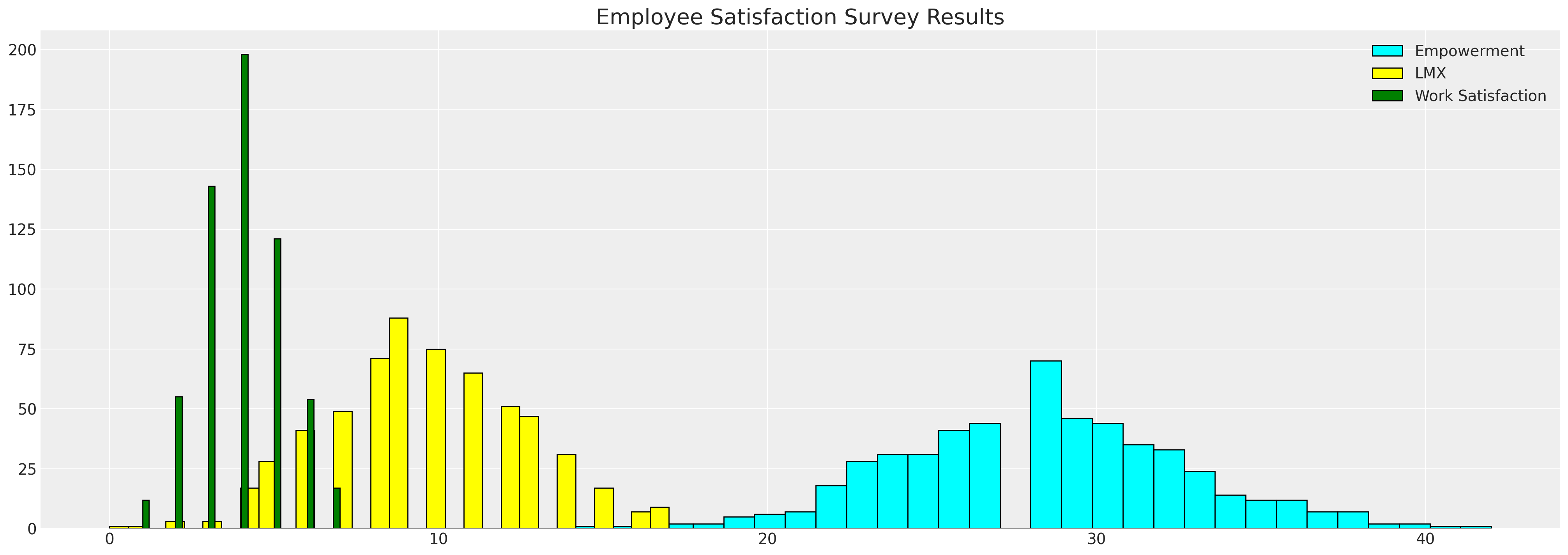We see here the histograms of the employee metrics. It is the gaps in the data that we wish to impute to better understand the relationships between the variables and how gaps in one may be driven by values in the others.

## FIML: Full Information Maximum Likelihood#

This method of handling missing data is not an imputation method. It uses maximum likelihood estimation to estimate the parameters of the multivariate normal distribution that could be best said to generate our observed data. It’s a little trickier than straight forward MLE approaches in that it respects the fact that we have missing data in our original data set, but fundamentally it’s the same idea. We want to optimize the parameters of our multivariate normal distribution to best fit the observed data.

The procedure works by partitioning the data into their patterns of “missing-ness” and treating each partition as contributing to the ultimate log-likelihood term that we want to maximise. We combine their contributions to estimate a fit for the multivariate normal distribution.

data = df_employee[["worksat", "empower", "lmx"]]

def split_data_by_missing_pattern(data):
# We want to extract our the pattern of missing-ness in our dataset
# and save each sub-set of our data in a structure that can be used to feed into a log-likelihood function
grouped_patterns = []
patterns = data.notnull().drop_duplicates().values
# A pattern is whether the values in each column e.g. [True, True, True] or [True, True, False]
observed = data.notnull()
for p in range(len(patterns)):
temp = observed[
(observed["worksat"] == patterns[p])
& (observed["empower"] == patterns[p])
& (observed["lmx"] == patterns[p])
]
grouped_patterns.append([patterns[p], temp.index, data.iloc[temp.index].dropna(axis=1)])

return grouped_patterns

def reconstitute_params(params_vector, n_vars):
# Convenience numpy function to construct mirrored COV matrix
# From flattened params_vector
mus = params_vector[0:n_vars]
cov_flat = params_vector[n_vars:]
indices = np.tril_indices(n_vars)
cov = np.empty((n_vars, n_vars))
for i, j, c in zip(indices, indices, cov_flat):
cov[i, j] = c
cov[j, i] = c
cov = cov + 1e-25
return mus, cov

def optimise_ll(flat_params, n_vars, grouped_patterns):
mus, cov = reconstitute_params(flat_params, n_vars)
# Check if COV is positive definite
if (np.linalg.eigvalsh(cov) < 0).any():
return 1e100
objval = 0.0
for obs_pattern, _, obs_data in grouped_patterns:
# This is the key (tricky) step because we're selecting the variables which pattern
# the full information set within each pattern of "missing-ness"
# e.g. when the observed pattern is [True, True, False] we want the first two variables
# of the mus vector and we want only the covariance relations between the relevant variables from the cov
# in the iteration.
obs_mus = mus[obs_pattern]
obs_cov = cov[obs_pattern][:, obs_pattern]
ll = np.sum(multivariate_normal(obs_mus, obs_cov).logpdf(obs_data))
objval = ll + objval
return -objval

def estimate(data):
n_vars = data.shape
# Initialise
mus0 = np.zeros(n_vars)
cov0 = np.eye(n_vars)
# Flatten params for optimiser
params0 = np.append(mus0, cov0[np.tril_indices(n_vars)])
# Process Data
grouped_patterns = split_data_by_missing_pattern(data)
# Run the Optimiser.
try:
result = scipy.optimize.minimize(
optimise_ll, params0, args=(n_vars, grouped_patterns), method="Powell"
)
except Exception as e:
raise e
mean, cov = reconstitute_params(result.x, n_vars)
return mean, cov

fiml_mus, fiml_cov = estimate(data)

print("Full information Maximum Likelihood Estimate Mu:")
display(pd.DataFrame(fiml_mus, index=data.columns).T)
print("Full information Maximum Likelihood Estimate COV:")
pd.DataFrame(fiml_cov, columns=data.columns, index=data.columns)

Full information Maximum Likelihood Estimate Mu:

worksat empower lmx
0 3.983351 28.595211 9.624485
Full information Maximum Likelihood Estimate COV:

worksat empower lmx
worksat 1.568676 1.599817 1.547433
empower 1.599817 19.138522 5.428954
lmx 1.547433 5.428954 8.934030

### Sampling from the Implied Distribution#

We can then sample from the implied distribution to estimate other features of interest and test against the observed data.

mle_fit = multivariate_normal(fiml_mus, fiml_cov)
mle_sample = mle_fit.rvs(10000)
mle_sample = pd.DataFrame(mle_sample, columns=["worksat", "empower", "lmx"])

worksat empower lmx
0 4.467296 31.568011 12.418765
1 4.713191 30.329419 10.651786
2 5.699765 35.770312 12.558135
3 4.067691 27.874578 6.271341
4 3.580109 28.799105 9.704713

This allows us to compare the implied distributions against the observed data

fig, ax = plt.subplots(figsize=(20, 7))
ax.hist(
mle_sample["empower"],
bins=30,
ec="black",
color="cyan",
alpha=0.2,
label="Inferred Empowerment",
)
ax.hist(mle_sample["lmx"], bins=30, ec="black", color="yellow", alpha=0.2, label="Inferred LMX")
ax.hist(
mle_sample["worksat"],
bins=30,
ec="black",
color="green",
alpha=0.2,
label="Inferred Work Satisfaction",
)
ax.hist(data["empower"], bins=30, ec="black", color="cyan", label="Observed Empowerment")
ax.hist(data["lmx"], bins=30, ec="black", color="yellow", label="Observed LMX")
ax.hist(data["worksat"], bins=30, ec="black", color="green", label="Observed Work Satisfaction")
ax.set_title("Inferred from MLE fit: Employee Satisfaction Survey Results", fontsize=20)
ax.legend()

<matplotlib.legend.Legend at 0x1914bce50>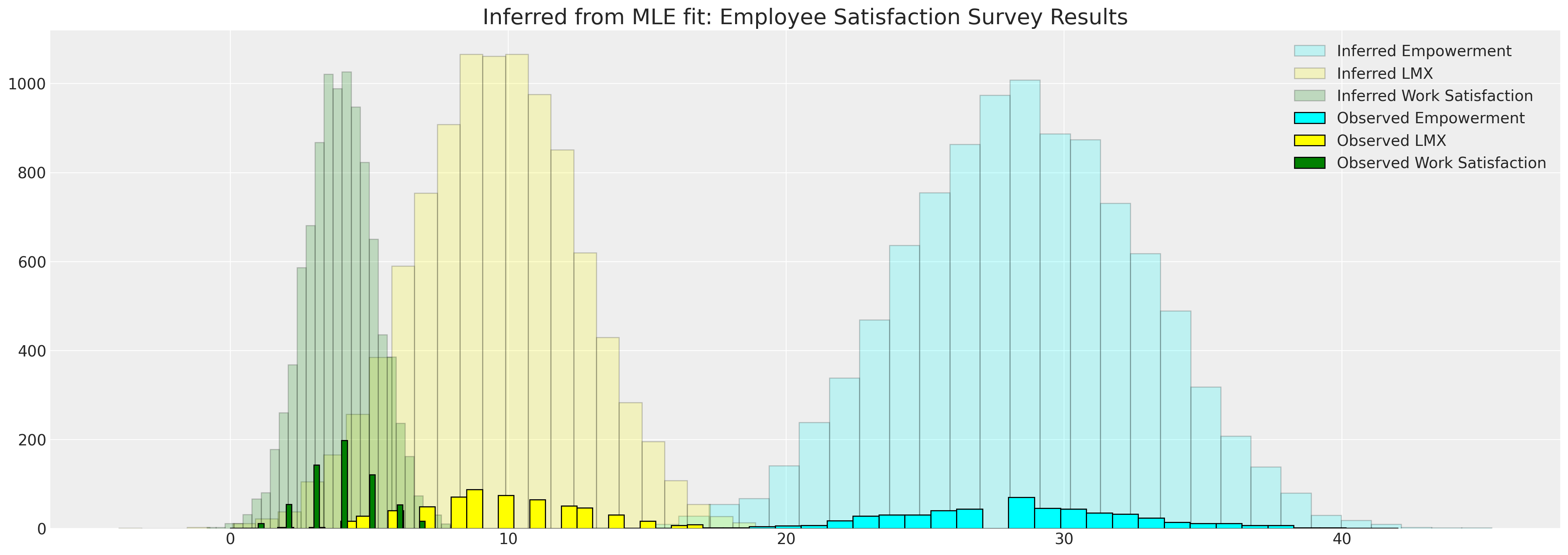### The Correlation Between the Imputed Metrics Data#

We can also calculate other features of interest from our sample. For instance, we might want to know about the correlations between variables in question.

pd.DataFrame(mle_sample.corr(), columns=data.columns, index=data.columns)

worksat empower lmx
worksat 1.000000 0.300790 0.409996
empower 0.300790 1.000000 0.410874
lmx 0.409996 0.410874 1.000000

### Bootstrapping Sensitivity Analysis#

We may also want to validate the estimated parameters against bootstrapped samples under different speficiations of missing-ness.

data_200 = df_employee[["worksat", "empower", "lmx"]].dropna().sample(200)
data_200.reset_index(inplace=True, drop=True)

sensitivity = {}
n_missing = np.linspace(30, 100, 5)  # Change or alter the range as desired
bootstrap_iterations = 100  # change to large number running a real analysis in this case
for n in n_missing:
sensitivity[int(n)] = {}
sensitivity[int(n)]["mus"] = []
sensitivity[int(n)]["cov"] = []
for i in range(bootstrap_iterations):
temp = data_200.copy()
for m in range(int(n)):
i = random.choice(range(200))
j = random.choice(range(3))
temp.iloc[i, j] = np.nan
try:
fiml_mus, fiml_cov = estimate(temp)
sensitivity[int(n)]["mus"].append(fiml_mus)
sensitivity[int(n)]["cov"].append(fiml_cov)
except Exception as e:
next


Here we plot the maximum likelihood parameter estimates against various missing data regimes. This approach can be applied for any imputation methodology.

fig, axs = plt.subplots(1, 3, figsize=(20, 7))
for n in sensitivity.keys():
temp = pd.DataFrame(sensitivity[n]["mus"], columns=["worksat", "empower", "lmx"])
for col, ax in zip(temp.columns, axs):
ax.hist(
temp[col], alpha=0.1, ec="black", label=f"Missing: {np.round(n/200, 2)}, Mean: {col}"
)
ax.legend()
ax.set_title(f"Bootstrap Distribution for Mean:\n{col}")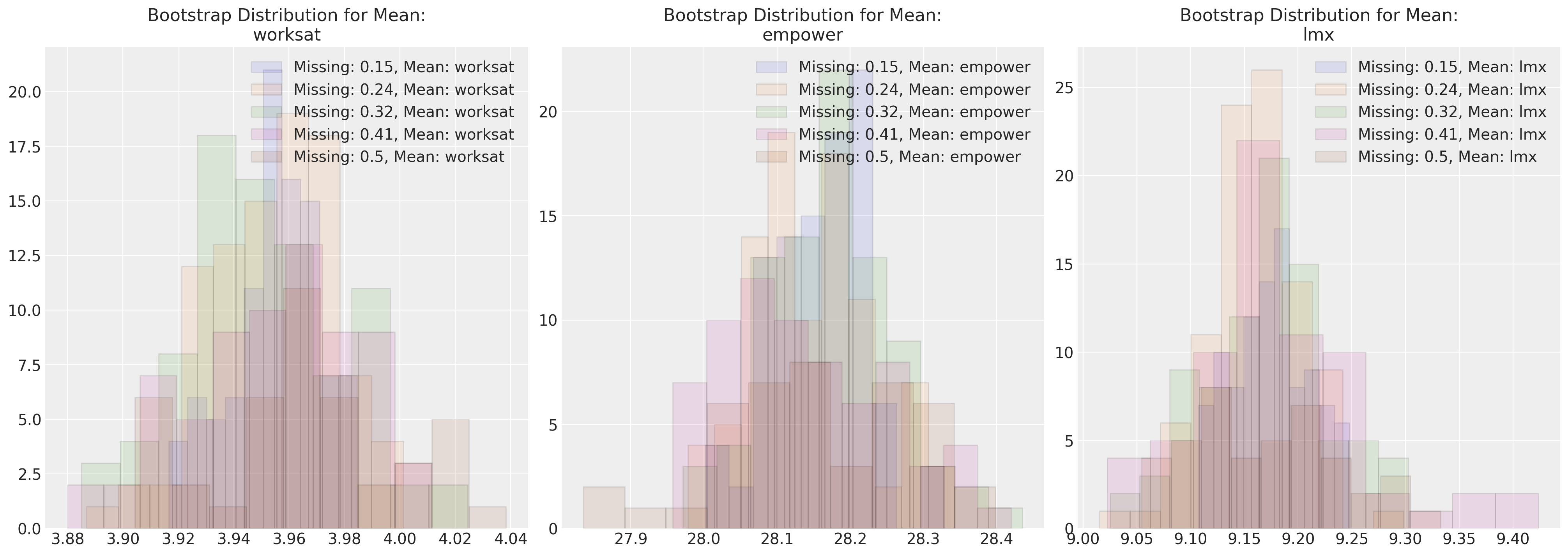fig, axs = plt.subplots(2, 3, figsize=(20, 14))
axs = axs.flatten()
for n in sensitivity.keys():
length = len(sensitivity[n]["cov"])
temp = pd.DataFrame(
[sensitivity[n]["cov"][i][np.tril_indices(3)] for i in range(length)],
columns=[
"var(worksat)",
"cov(worksat, empower)",
"var(empower)",
"cov(worksat, lmx)",
"cov(lmx, empower)",
"var(lmx)",
],
)
for col, ax in zip(temp.columns, axs):
ax.hist(
temp[col], alpha=0.1, ec="black", label=f"Missing: {np.round(n/200, 2)}, Mean: {col}"
)
ax.legend()
ax.set_title(f"Bootstrap Distribution for Expected:\n{col}")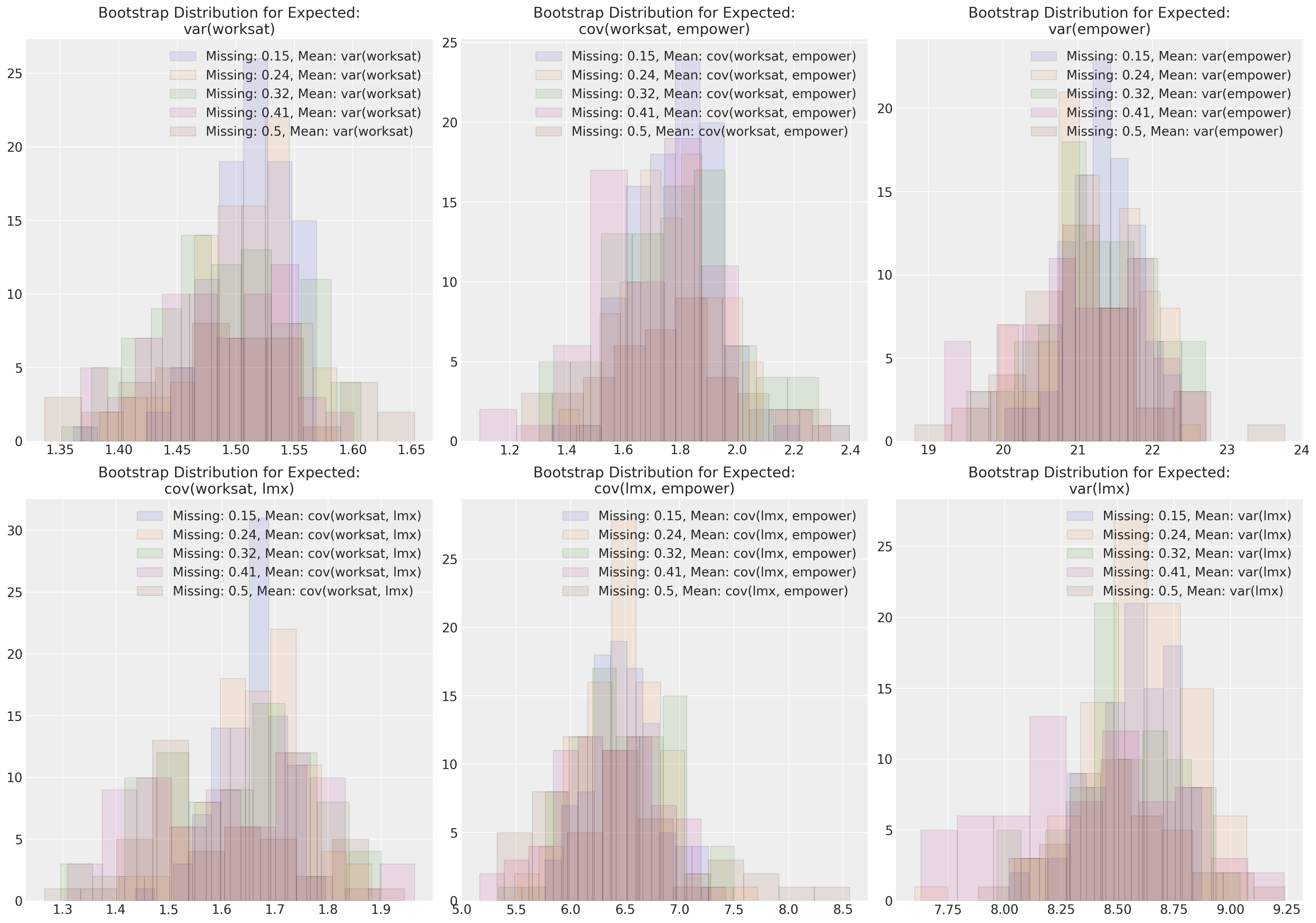These plots show how under (MCAR) the parameter estimates of our multivariate normal distribution are quite robust to varying degrees of missing data. It’s an instructive exercise to attempt a similar simulation exercise under alternative missing data regimes.

## Bayesian Imputation#

Next we’ll apply bayesian methods to the same problem. But here we’ll see direct imputation of the missing values using the posterior predictive distribution. The Bayesian approach to imputation is of a different flavour than we saw above. We’re not just learning parameters of the data generating distribution (although we are doing that too), the bayesian process directly imputes the missing values for specific missing entries through the process of MCMC sampling.

import pytensor.tensor as pt

with pm.Model() as model:
# Priors
mus = pm.Normal("mus", 0, 1, size=3)
cov_flat_prior, _, _ = pm.LKJCholeskyCov("cov", n=3, eta=1.0, sd_dist=pm.Exponential.dist(1))
# Create a vector of flat variables for the unobserved components of the MvNormal
x_unobs = pm.Uniform("x_unobs", 0, 100, shape=(np.isnan(data.values).sum(),))

# Create the symbolic value of x, combining observed data and unobserved variables
x = pt.as_tensor(data.values)
x = pm.Deterministic("x", pt.set_subtensor(x[np.isnan(data.values)], x_unobs))

# Add a Potential with the logp of the variable conditioned on x
pm.Potential("x_logp", pm.logp(rv=pm.MvNormal.dist(mus, chol=cov_flat_prior), value=x))
idata = pm.sample_prior_predictive()
idata = pm.sample()
idata.extend(pm.sample(random_seed=120))
pm.sample_posterior_predictive(idata, extend_inferencedata=True)

pm.model_to_graphviz(model)

/var/folders/99/gp2xl6x513s0tvl3cx79zf7m0000gn/T/ipykernel_96943/3865616598.py:16: UserWarning: The effect of Potentials on other parameters is ignored during prior predictive sampling. This is likely to lead to invalid or biased predictive samples.
idata = pm.sample_prior_predictive()
Sampling: [cov, mus, x_unobs]
Auto-assigning NUTS sampler...
Multiprocess sampling (4 chains in 4 jobs)
NUTS: [mus, cov, x_unobs]

100.00% [8000/8000 01:07<00:00 Sampling 4 chains, 0 divergences]
/Users/nathanielforde/opt/miniconda3/envs/missing_data_clean/lib/python3.11/site-packages/pytensor/compile/function/types.py:972: RuntimeWarning: invalid value encountered in accumulate
self.vm()
Sampling 4 chains for 1_000 tune and 1_000 draw iterations (4_000 + 4_000 draws total) took 98 seconds.
Auto-assigning NUTS sampler...
Multiprocess sampling (4 chains in 4 jobs)
NUTS: [mus, cov, x_unobs]

100.00% [8000/8000 01:06<00:00 Sampling 4 chains, 0 divergences]
/Users/nathanielforde/opt/miniconda3/envs/missing_data_clean/lib/python3.11/site-packages/pytensor/compile/function/types.py:972: RuntimeWarning: invalid value encountered in accumulate
self.vm()
/Users/nathanielforde/opt/miniconda3/envs/missing_data_clean/lib/python3.11/site-packages/pytensor/compile/function/types.py:972: RuntimeWarning: invalid value encountered in accumulate
self.vm()
Sampling 4 chains for 1_000 tune and 1_000 draw iterations (4_000 + 4_000 draws total) took 99 seconds.
/var/folders/99/gp2xl6x513s0tvl3cx79zf7m0000gn/T/ipykernel_96943/3865616598.py:19: UserWarning: The effect of Potentials on other parameters is ignored during posterior predictive sampling. This is likely to lead to invalid or biased predictive samples.
pm.sample_posterior_predictive(idata, extend_inferencedata=True)az.plot_posterior(idata, var_names=["mus", "cov"]);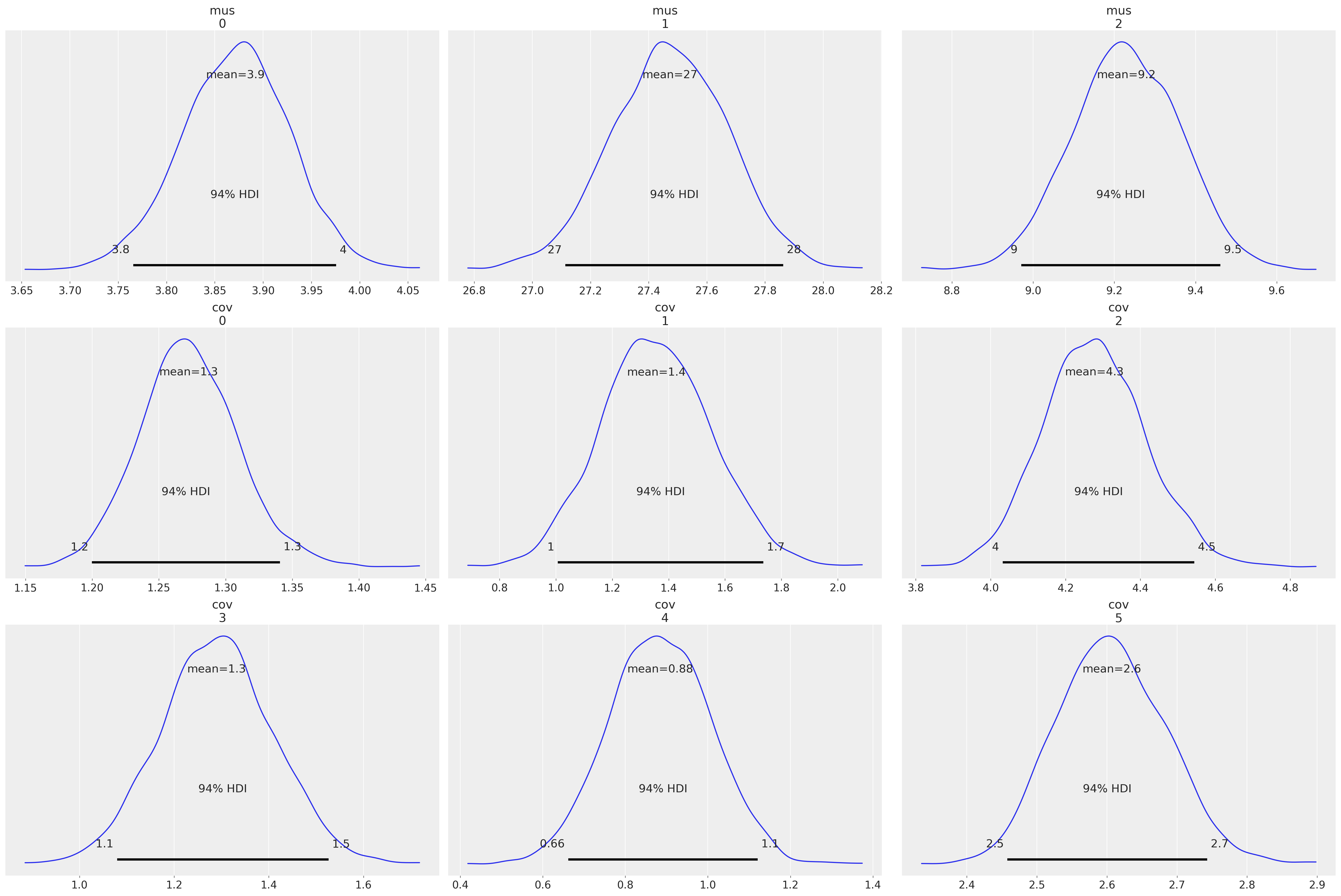az.summary(idata, var_names=["mus", "cov", "x_unobs"])

mean sd hdi_3% hdi_97% mcse_mean mcse_sd ess_bulk ess_tail r_hat
mus 3.871 0.056 3.766 3.976 0.001 0.001 6110.0 3277.0 1.0
mus 27.473 0.200 27.114 27.863 0.003 0.002 5742.0 3320.0 1.0
mus 9.229 0.132 8.971 9.461 0.002 0.001 6154.0 3271.0 1.0
cov 1.272 0.037 1.200 1.341 0.000 0.000 6235.0 2754.0 1.0
cov 1.356 0.197 1.007 1.736 0.003 0.002 5373.0 3750.0 1.0
... ... ... ... ... ... ... ... ... ...
x_unobs 29.836 4.205 21.820 37.745 0.044 0.031 9232.0 2929.0 1.0
x_unobs 2.559 1.107 0.356 4.483 0.018 0.013 3564.0 1634.0 1.0
x_unobs 30.071 4.029 22.614 37.652 0.039 0.028 10697.0 3078.0 1.0
x_unobs 29.654 4.017 22.079 37.411 0.039 0.027 10626.0 2867.0 1.0
x_unobs 27.420 4.066 19.595 34.915 0.046 0.033 7784.0 2226.0 1.0

167 rows × 9 columns

imputed_dims = data.shape
imputed = data.values.flatten()
imputed[np.isnan(imputed)] = az.summary(idata, var_names=["x_unobs"])["mean"].values
imputed = imputed.reshape(imputed_dims, imputed_dims)
imputed = pd.DataFrame(imputed, columns=[col + "_imputed" for col in data.columns])

worksat_imputed empower_imputed lmx_imputed
0 3.000 32.000 11.000
1 4.000 29.431 13.000
2 4.000 30.000 9.000
3 3.000 29.000 8.000
4 4.000 26.000 7.000
5 3.995 27.915 10.000
6 5.000 28.984 11.000
7 3.000 22.000 9.000
8 2.000 23.000 6.835
9 4.000 32.000 9.000
fig, axs = plt.subplots(1, 3, figsize=(20, 7))
axs = axs.flatten()
for col, col_i, ax in zip(data.columns, imputed.columns, axs):
ax.hist(data[col], color="red", label=col, ec="black", bins=30)
ax.hist(imputed[col_i], color="cyan", alpha=0.3, label=col_i, ec="black", bins=30)
ax.legend()
ax.set_title(f"Imputed Distribution and Observed for {col}")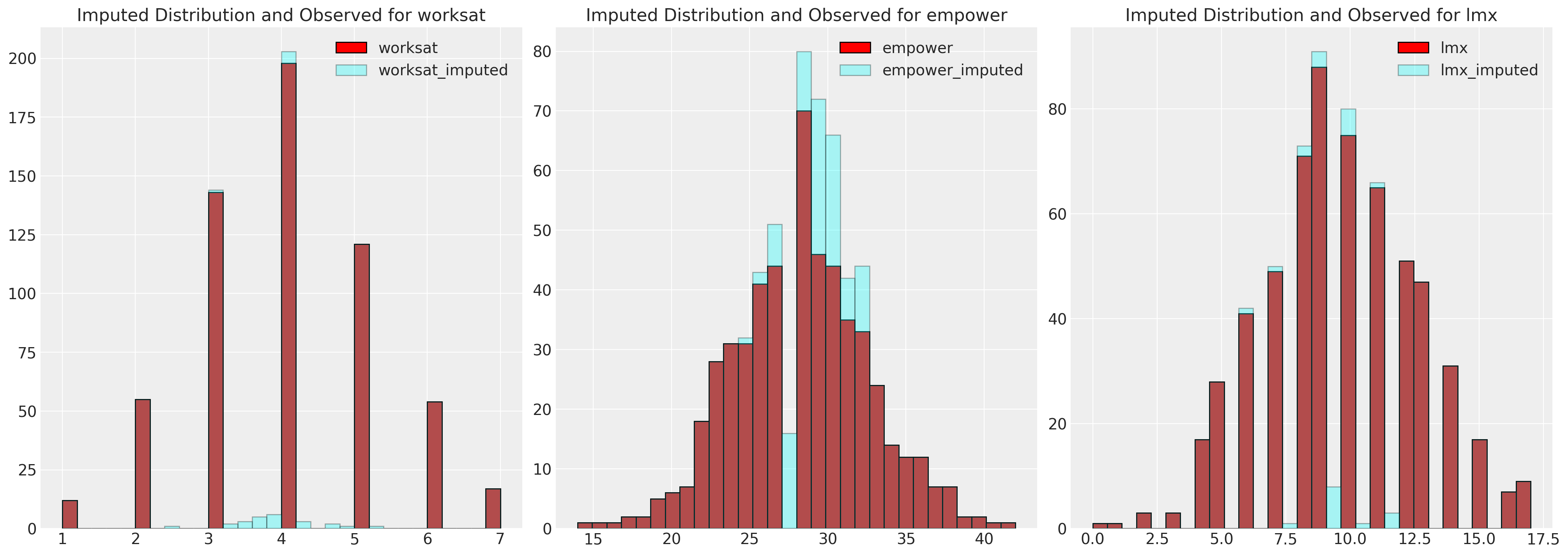pd.DataFrame(az.summary(idata, var_names=["cov_corr"])["mean"].values.reshape(3, 3))

/Users/nathanielforde/opt/miniconda3/envs/missing_data_clean/lib/python3.11/site-packages/arviz/stats/diagnostics.py:584: RuntimeWarning: invalid value encountered in scalar divide
(between_chain_variance / within_chain_variance + num_samples - 1) / (num_samples)

0 1 2
0 1.000 0.302 0.423
1 0.302 1.000 0.405
2 0.423 0.405 1.000

These results agree with the FIML approach above and the results reported in Ender’s Applied Missing Data Analysis.

## Bayesian Imputation by Chained Equations#

So far we’ve seen multivariate approaches to imputation which treat each of the variables in our dataset as a collection drawn from the same distribution. However, there is a more flexible approach which is often useful when there is a particular focal relationship that we’re interested in analysing.

Sticking with the employee data set we’ll examine here the relationship between lmx, climate, male and empower, where our focus is on what drives empowerment. Recall that our gender variable male is fully specified and does not need to be imputed. So we have a joint distribution that can be decomposed:

$f(emp, lmx, climate, male) = f(emp | lmx, climate, male) \cdot f(lmx | climate, male) \cdot f(climate | male) \cdot f(male)^{*}$

which can be split out into individual regression equations or more generally component models for each required conditional model.

$empower = \alpha_{2} + \beta_{3}male + \beta_{4}climate + \beta_{5}lmx$
$lmx = \alpha_{1} + \beta_{1}climate + \beta_{2}male$
$climate = \alpha_{0} + \beta_{0}male$

We can impute each of these equations in turn saving the imputed data set and feeding it forward into the next modelling exercise. This adds a little complexity because some of the variables will occur twice. Once as a predictor in our focal regression and once and as likelihood term in their own component model.

### PyMC Imputation#

As we saw above we can use PyMC to impute the values of missing data by using a particular sampling distribution. In the case of chained equations this becomes a little trickier because we might want to use both the data for lmx as a regressor in one equation and observed data in our likelihood in another.

It also matters how we specify the sampling distribution that will be used to impute our missing data. We’ll show an example here where we use a uniform and normal sampling distribution alternatively for imputing the predictor terms in our in focal regression.

data = df_employee[["lmx", "empower", "climate", "male"]]
lmx_mean = data["lmx"].mean()
lmx_min = data["lmx"].min()
lmx_max = data["lmx"].max()
lmx_sd = data["lmx"].std()

cli_mean = data["climate"].mean()
cli_min = data["climate"].min()
cli_max = data["climate"].max()
cli_sd = data["climate"].std()

priors = {
"climate": {"normal": [lmx_mean, lmx_sd, lmx_sd], "uniform": [lmx_min, lmx_max]},
"lmx": {"normal": [cli_mean, cli_sd, cli_sd], "uniform": [cli_min, cli_max]},
}

def make_model(priors, normal_pred_assumption=True):
coords = {
"alpha_dim": ["lmx_imputed", "climate_imputed", "empower_imputed"],
"beta_dim": [
"lmxB_male",
"lmxB_climate",
"climateB_male",
"empB_male",
"empB_climate",
"empB_lmx",
],
}
with pm.Model(coords=coords) as model:
# Priors
beta = pm.Normal("beta", 0, 1, size=6, dims="beta_dim")
alpha = pm.Normal("alphas", 10, 5, size=3, dims="alpha_dim")
sigma = pm.HalfNormal("sigmas", 5, size=3, dims="alpha_dim")

if normal_pred_assumption:
mu_climate = pm.Normal(
"mu_climate", priors["climate"]["normal"], priors["climate"]["normal"]
)
sigma_climate = pm.HalfNormal("sigma_climate", priors["climate"]["normal"])
climate_pred = pm.Normal(
"climate_pred", mu_climate, sigma_climate, observed=data["climate"].values
)
else:
climate_pred = pm.Uniform("climate_pred", 0, 40, observed=data["climate"].values)

if normal_pred_assumption:
mu_lmx = pm.Normal("mu_lmx", priors["lmx"]["normal"], priors["lmx"]["normal"])
sigma_lmx = pm.HalfNormal("sigma_lmx", priors["lmx"]["normal"])
lmx_pred = pm.Normal("lmx_pred", mu_lmx, sigma_lmx, observed=data["lmx"].values)
else:
lmx_pred = pm.Uniform("lmx_pred", 0, 40, observed=data["lmx"].values)

# Likelihood(s)
lmx_imputed = pm.Normal(
"lmx_imputed",
alpha + beta * data["male"] + beta * climate_pred,
sigma,
observed=data["lmx"].values,
)
climate_imputed = pm.Normal(
"climate_imputed",
alpha + beta * data["male"],
sigma,
observed=data["climate"].values,
)
empower_imputed = pm.Normal(
"emp_imputed",
alpha + beta * data["male"] + beta * climate_pred + beta * lmx_pred,
sigma,
observed=data["empower"].values,
)

idata = pm.sample_prior_predictive()
idata.extend(pm.sample(random_seed=120))
pm.sample_posterior_predictive(idata, extend_inferencedata=True)
return idata, model

idata_uniform, model_uniform = make_model(priors, normal_pred_assumption=False)
idata_normal, model_normal = make_model(priors, normal_pred_assumption=True)
pm.model_to_graphviz(model_uniform)

/Users/nathanielforde/opt/miniconda3/envs/missing_data_clean/lib/python3.11/site-packages/pymc/model.py:1400: ImputationWarning: Data in climate_pred contains missing values and will be automatically imputed from the sampling distribution.
warnings.warn(impute_message, ImputationWarning)
/Users/nathanielforde/opt/miniconda3/envs/missing_data_clean/lib/python3.11/site-packages/pymc/model.py:1400: ImputationWarning: Data in lmx_pred contains missing values and will be automatically imputed from the sampling distribution.
warnings.warn(impute_message, ImputationWarning)
/Users/nathanielforde/opt/miniconda3/envs/missing_data_clean/lib/python3.11/site-packages/pymc/model.py:1400: ImputationWarning: Data in lmx_imputed contains missing values and will be automatically imputed from the sampling distribution.
warnings.warn(impute_message, ImputationWarning)
/Users/nathanielforde/opt/miniconda3/envs/missing_data_clean/lib/python3.11/site-packages/pymc/model.py:1400: ImputationWarning: Data in climate_imputed contains missing values and will be automatically imputed from the sampling distribution.
warnings.warn(impute_message, ImputationWarning)
/Users/nathanielforde/opt/miniconda3/envs/missing_data_clean/lib/python3.11/site-packages/pymc/model.py:1400: ImputationWarning: Data in emp_imputed contains missing values and will be automatically imputed from the sampling distribution.
warnings.warn(impute_message, ImputationWarning)
Sampling: [alphas, beta, climate_imputed_missing, climate_imputed_observed, climate_pred_missing, climate_pred_observed, emp_imputed_missing, emp_imputed_observed, lmx_imputed_missing, lmx_imputed_observed, lmx_pred_missing, lmx_pred_observed, sigmas]
Auto-assigning NUTS sampler...
Multiprocess sampling (4 chains in 4 jobs)
NUTS: [beta, alphas, sigmas, climate_pred_missing, lmx_pred_missing, lmx_imputed_missing, climate_imputed_missing, emp_imputed_missing]

100.00% [8000/8000 01:08<00:00 Sampling 4 chains, 0 divergences]
Sampling 4 chains for 1_000 tune and 1_000 draw iterations (4_000 + 4_000 draws total) took 96 seconds.
Sampling: [climate_imputed_observed, climate_pred_observed, emp_imputed_missing, emp_imputed_observed, lmx_imputed_missing, lmx_imputed_observed, lmx_pred_observed]

100.00% [4000/4000 00:01<00:00]
/Users/nathanielforde/opt/miniconda3/envs/missing_data_clean/lib/python3.11/site-packages/pymc/model.py:1400: ImputationWarning: Data in climate_pred contains missing values and will be automatically imputed from the sampling distribution.
warnings.warn(impute_message, ImputationWarning)
/Users/nathanielforde/opt/miniconda3/envs/missing_data_clean/lib/python3.11/site-packages/pymc/model.py:1400: ImputationWarning: Data in lmx_pred contains missing values and will be automatically imputed from the sampling distribution.
warnings.warn(impute_message, ImputationWarning)
/Users/nathanielforde/opt/miniconda3/envs/missing_data_clean/lib/python3.11/site-packages/pymc/model.py:1400: ImputationWarning: Data in lmx_imputed contains missing values and will be automatically imputed from the sampling distribution.
warnings.warn(impute_message, ImputationWarning)
/Users/nathanielforde/opt/miniconda3/envs/missing_data_clean/lib/python3.11/site-packages/pymc/model.py:1400: ImputationWarning: Data in climate_imputed contains missing values and will be automatically imputed from the sampling distribution.
warnings.warn(impute_message, ImputationWarning)
/Users/nathanielforde/opt/miniconda3/envs/missing_data_clean/lib/python3.11/site-packages/pymc/model.py:1400: ImputationWarning: Data in emp_imputed contains missing values and will be automatically imputed from the sampling distribution.
warnings.warn(impute_message, ImputationWarning)
Sampling: [alphas, beta, climate_imputed_missing, climate_imputed_observed, climate_pred_missing, climate_pred_observed, emp_imputed_missing, emp_imputed_observed, lmx_imputed_missing, lmx_imputed_observed, lmx_pred_missing, lmx_pred_observed, mu_climate, mu_lmx, sigma_climate, sigma_lmx, sigmas]
Auto-assigning NUTS sampler...
Multiprocess sampling (4 chains in 4 jobs)
NUTS: [beta, alphas, sigmas, mu_climate, sigma_climate, climate_pred_missing, mu_lmx, sigma_lmx, lmx_pred_missing, lmx_imputed_missing, climate_imputed_missing, emp_imputed_missing]

100.00% [8000/8000 01:15<00:00 Sampling 4 chains, 0 divergences]
Sampling 4 chains for 1_000 tune and 1_000 draw iterations (4_000 + 4_000 draws total) took 106 seconds.
Sampling: [climate_imputed_observed, climate_pred_observed, emp_imputed_missing, emp_imputed_observed, lmx_imputed_missing, lmx_imputed_observed, lmx_pred_observed]

100.00% [4000/4000 00:01<00:00]idata_normal

arviz.InferenceData
• <xarray.Dataset>
Dimensions:                        (chain: 4, draw: 1000, beta_dim: 6,
alpha_dim: 3,
climate_pred_missing_dim_0: 60,
lmx_pred_missing_dim_0: 26,
lmx_imputed_missing_dim_0: 26,
climate_imputed_missing_dim_0: 60,
emp_imputed_missing_dim_0: 102,
climate_pred_dim_0: 630,
lmx_pred_dim_0: 630,
lmx_imputed_dim_0: 630,
climate_imputed_dim_0: 630,
emp_imputed_dim_0: 630)
Coordinates: (12/14)
* chain                          (chain) int64 0 1 2 3
* draw                           (draw) int64 0 1 2 3 4 ... 996 997 998 999
* beta_dim                       (beta_dim) <U13 'lmxB_male' ... 'empB_lmx'
* alpha_dim                      (alpha_dim) <U15 'lmx_imputed' ... 'empowe...
* climate_pred_missing_dim_0     (climate_pred_missing_dim_0) int64 0 1 ... 59
* lmx_pred_missing_dim_0         (lmx_pred_missing_dim_0) int64 0 1 ... 24 25
...                             ...
* emp_imputed_missing_dim_0      (emp_imputed_missing_dim_0) int64 0 1 ... 101
* climate_pred_dim_0             (climate_pred_dim_0) int64 0 1 2 ... 628 629
* lmx_pred_dim_0                 (lmx_pred_dim_0) int64 0 1 2 ... 627 628 629
* lmx_imputed_dim_0              (lmx_imputed_dim_0) int64 0 1 2 ... 628 629
* climate_imputed_dim_0          (climate_imputed_dim_0) int64 0 1 ... 628 629
* emp_imputed_dim_0              (emp_imputed_dim_0) int64 0 1 2 ... 628 629
Data variables: (12/17)
beta                           (chain, draw, beta_dim) float64 0.5683 ......
alphas                         (chain, draw, alpha_dim) float64 9.008 ......
mu_climate                     (chain, draw) float64 19.98 20.11 ... 20.12
climate_pred_missing           (chain, draw, climate_pred_missing_dim_0) float64 ...
mu_lmx                         (chain, draw) float64 9.514 9.723 ... 9.586
lmx_pred_missing               (chain, draw, lmx_pred_missing_dim_0) float64 ...
...                             ...
sigma_lmx                      (chain, draw) float64 3.027 3.152 ... 3.004
climate_pred                   (chain, draw, climate_pred_dim_0) float64 ...
lmx_pred                       (chain, draw, lmx_pred_dim_0) float64 11.0...
lmx_imputed                    (chain, draw, lmx_imputed_dim_0) float64 1...
climate_imputed                (chain, draw, climate_imputed_dim_0) float64 ...
emp_imputed                    (chain, draw, emp_imputed_dim_0) float64 3...
Attributes:
created_at:                 2023-02-02T07:57:06.498924
arviz_version:              0.14.0
inference_library:          pymc
inference_library_version:  5.0.1
sampling_time:              106.22190403938293
tuning_steps:               1000

• <xarray.Dataset>
Dimensions:                         (chain: 4, draw: 1000,
climate_pred_observed_dim_2: 570,
lmx_pred_observed_dim_2: 604,
lmx_imputed_observed_dim_2: 604,
climate_imputed_observed_dim_2: 570,
emp_imputed_observed_dim_2: 528,
climate_pred_dim_2: 630,
lmx_pred_dim_2: 630,
lmx_imputed_dim_2: 630,
climate_imputed_dim_2: 630,
emp_imputed_dim_2: 630)
Coordinates:
* chain                           (chain) int64 0 1 2 3
* draw                            (draw) int64 0 1 2 3 4 ... 996 997 998 999
* climate_pred_observed_dim_2     (climate_pred_observed_dim_2) int64 0 ......
* lmx_pred_observed_dim_2         (lmx_pred_observed_dim_2) int64 0 1 ... 603
* lmx_imputed_observed_dim_2      (lmx_imputed_observed_dim_2) int64 0 ... 603
* climate_imputed_observed_dim_2  (climate_imputed_observed_dim_2) int64 0 ...
* emp_imputed_observed_dim_2      (emp_imputed_observed_dim_2) int64 0 ... 527
* climate_pred_dim_2              (climate_pred_dim_2) int64 0 1 2 ... 628 629
* lmx_pred_dim_2                  (lmx_pred_dim_2) int64 0 1 2 ... 627 628 629
* lmx_imputed_dim_2               (lmx_imputed_dim_2) int64 0 1 2 ... 628 629
* climate_imputed_dim_2           (climate_imputed_dim_2) int64 0 1 ... 629
* emp_imputed_dim_2               (emp_imputed_dim_2) int64 0 1 2 ... 628 629
Data variables:
climate_pred_observed           (chain, draw, climate_pred_observed_dim_2) float64 ...
lmx_pred_observed               (chain, draw, lmx_pred_observed_dim_2) float64 ...
lmx_imputed_observed            (chain, draw, lmx_imputed_observed_dim_2) float64 ...
climate_imputed_observed        (chain, draw, climate_imputed_observed_dim_2) float64 ...
emp_imputed_observed            (chain, draw, emp_imputed_observed_dim_2) float64 ...
climate_pred                    (chain, draw, climate_pred_dim_2) float64 ...
lmx_pred                        (chain, draw, lmx_pred_dim_2) float64 8.6...
lmx_imputed                     (chain, draw, lmx_imputed_dim_2) float64 ...
climate_imputed                 (chain, draw, climate_imputed_dim_2) float64 ...
emp_imputed                     (chain, draw, emp_imputed_dim_2) float64 ...
Attributes:
created_at:                 2023-02-02T07:57:11.095286
arviz_version:              0.14.0
inference_library:          pymc
inference_library_version:  5.0.1

• <xarray.Dataset>
Dimensions:                (chain: 4, draw: 1000)
Coordinates:
* chain                  (chain) int64 0 1 2 3
* draw                   (draw) int64 0 1 2 3 4 5 ... 994 995 996 997 998 999
Data variables: (12/17)
n_steps                (chain, draw) float64 31.0 31.0 31.0 ... 31.0 31.0
max_energy_error       (chain, draw) float64 -0.3783 -0.1605 ... 0.6239
diverging              (chain, draw) bool False False False ... False False
reached_max_treedepth  (chain, draw) bool False False False ... False False
acceptance_rate        (chain, draw) float64 0.9975 0.9587 ... 0.6311 0.7695
process_time_diff      (chain, draw) float64 0.02338 0.02421 ... 0.01917
...                     ...
perf_counter_start     (chain, draw) float64 4.427e+05 ... 4.427e+05
energy                 (chain, draw) float64 8.642e+03 ... 8.615e+03
lp                     (chain, draw) float64 -8.501e+03 ... -8.471e+03
energy_error           (chain, draw) float64 -0.1605 0.1162 ... -0.08054
largest_eigval         (chain, draw) float64 nan nan nan nan ... nan nan nan
tree_depth             (chain, draw) int64 5 5 5 5 5 5 5 5 ... 5 5 5 5 5 5 5
Attributes:
created_at:                 2023-02-02T07:57:06.518637
arviz_version:              0.14.0
inference_library:          pymc
inference_library_version:  5.0.1
sampling_time:              106.22190403938293
tuning_steps:               1000

• <xarray.Dataset>
Dimensions:                        (chain: 1, draw: 500, alpha_dim: 3,
beta_dim: 6,
climate_pred_missing_dim_0: 60,
climate_imputed_missing_dim_0: 60,
emp_imputed_dim_0: 630,
climate_imputed_dim_0: 630,
lmx_pred_dim_0: 630,
lmx_imputed_missing_dim_0: 26,
emp_imputed_missing_dim_0: 102,
lmx_pred_missing_dim_0: 26,
lmx_imputed_dim_0: 630,
climate_pred_dim_0: 630)
Coordinates: (12/14)
* chain                          (chain) int64 0
* draw                           (draw) int64 0 1 2 3 4 ... 496 497 498 499
* alpha_dim                      (alpha_dim) <U15 'lmx_imputed' ... 'empowe...
* beta_dim                       (beta_dim) <U13 'lmxB_male' ... 'empB_lmx'
* climate_pred_missing_dim_0     (climate_pred_missing_dim_0) int64 0 1 ... 59
* climate_imputed_missing_dim_0  (climate_imputed_missing_dim_0) int64 0 .....
...                             ...
* lmx_pred_dim_0                 (lmx_pred_dim_0) int64 0 1 2 ... 627 628 629
* lmx_imputed_missing_dim_0      (lmx_imputed_missing_dim_0) int64 0 1 ... 25
* emp_imputed_missing_dim_0      (emp_imputed_missing_dim_0) int64 0 1 ... 101
* lmx_pred_missing_dim_0         (lmx_pred_missing_dim_0) int64 0 1 ... 24 25
* lmx_imputed_dim_0              (lmx_imputed_dim_0) int64 0 1 2 ... 628 629
* climate_pred_dim_0             (climate_pred_dim_0) int64 0 1 2 ... 628 629
Data variables: (12/17)
alphas                         (chain, draw, alpha_dim) float64 11.45 ......
sigma_climate                  (chain, draw) float64 1.15 0.4145 ... 0.8882
beta                           (chain, draw, beta_dim) float64 1.199 ... ...
climate_pred_missing           (chain, draw, climate_pred_missing_dim_0) float64 ...
climate_imputed_missing        (chain, draw, climate_imputed_missing_dim_0) float64 ...
emp_imputed                    (chain, draw, emp_imputed_dim_0) float64 8...
...                             ...
sigmas                         (chain, draw, alpha_dim) float64 6.3 ... 1...
lmx_pred_missing               (chain, draw, lmx_pred_missing_dim_0) float64 ...
sigma_lmx                      (chain, draw) float64 1.127 5.054 ... 6.724
lmx_imputed                    (chain, draw, lmx_imputed_dim_0) float64 2...
mu_climate                     (chain, draw) float64 4.559 9.647 ... 9.476
climate_pred                   (chain, draw, climate_pred_dim_0) float64 ...
Attributes:
created_at:                 2023-02-02T07:54:57.199499
arviz_version:              0.14.0
inference_library:          pymc
inference_library_version:  5.0.1

• <xarray.Dataset>
Dimensions:                         (chain: 1, draw: 500,
lmx_pred_observed_dim_0: 604,
emp_imputed_observed_dim_0: 528,
lmx_imputed_observed_dim_0: 604,
climate_imputed_observed_dim_0: 570,
climate_pred_observed_dim_0: 570)
Coordinates:
* chain                           (chain) int64 0
* draw                            (draw) int64 0 1 2 3 4 ... 496 497 498 499
* lmx_pred_observed_dim_0         (lmx_pred_observed_dim_0) int64 0 1 ... 603
* emp_imputed_observed_dim_0      (emp_imputed_observed_dim_0) int64 0 ... 527
* lmx_imputed_observed_dim_0      (lmx_imputed_observed_dim_0) int64 0 ... 603
* climate_imputed_observed_dim_0  (climate_imputed_observed_dim_0) int64 0 ...
* climate_pred_observed_dim_0     (climate_pred_observed_dim_0) int64 0 ......
Data variables:
lmx_pred_observed               (chain, draw, lmx_pred_observed_dim_0) float64 ...
emp_imputed_observed            (chain, draw, emp_imputed_observed_dim_0) float64 ...
lmx_imputed_observed            (chain, draw, lmx_imputed_observed_dim_0) float64 ...
climate_imputed_observed        (chain, draw, climate_imputed_observed_dim_0) float64 ...
climate_pred_observed           (chain, draw, climate_pred_observed_dim_0) float64 ...
Attributes:
created_at:                 2023-02-02T07:54:57.206651
arviz_version:              0.14.0
inference_library:          pymc
inference_library_version:  5.0.1

• <xarray.Dataset>
Dimensions:                         (climate_pred_observed_dim_0: 570,
lmx_pred_observed_dim_0: 604,
lmx_imputed_observed_dim_0: 604,
climate_imputed_observed_dim_0: 570,
emp_imputed_observed_dim_0: 528)
Coordinates:
* climate_pred_observed_dim_0     (climate_pred_observed_dim_0) int64 0 ......
* lmx_pred_observed_dim_0         (lmx_pred_observed_dim_0) int64 0 1 ... 603
* lmx_imputed_observed_dim_0      (lmx_imputed_observed_dim_0) int64 0 ... 603
* climate_imputed_observed_dim_0  (climate_imputed_observed_dim_0) int64 0 ...
* emp_imputed_observed_dim_0      (emp_imputed_observed_dim_0) int64 0 ... 527
Data variables:
climate_pred_observed           (climate_pred_observed_dim_0) float64 18....
lmx_pred_observed               (lmx_pred_observed_dim_0) float64 11.0 .....
lmx_imputed_observed            (lmx_imputed_observed_dim_0) float64 11.0...
climate_imputed_observed        (climate_imputed_observed_dim_0) float64 ...
emp_imputed_observed            (emp_imputed_observed_dim_0) float64 32.0...
Attributes:
created_at:                 2023-02-02T07:54:57.209280
arviz_version:              0.14.0
inference_library:          pymc
inference_library_version:  5.0.1

### Model Fits#

Next we’ll inspect the parameter fits for our regression models and observe how they’re dependent on the prior specification in the imputation scheme.

az.summary(idata_normal, var_names=["alphas", "beta", "sigmas"], stat_focus="median")

median mad eti_3% eti_97% mcse_median ess_median ess_tail r_hat
alphas[lmx_imputed] 9.057 0.446 7.854 10.263 0.011 3920.446 3077.0 1.00
alphas[climate_imputed] 19.776 0.158 19.345 20.213 0.005 4203.071 3452.0 1.00
alphas[empower_imputed] 17.928 0.689 16.016 19.851 0.022 3143.699 3063.0 1.00
beta[lmxB_male] 0.437 0.157 -0.005 0.894 0.003 7104.804 3102.0 1.00
beta[lmxB_climate] 0.018 0.022 -0.042 0.076 0.001 3670.069 2911.0 1.00
beta[climateB_male] 0.696 0.214 0.092 1.286 0.006 4471.550 3328.0 1.00
beta[empB_male] 1.656 0.214 1.043 2.254 0.005 5282.112 3361.0 1.00
beta[empB_climate] 0.203 0.030 0.121 0.286 0.001 3395.600 3068.0 1.00
beta[empB_lmx] 0.598 0.039 0.489 0.710 0.001 4541.732 2991.0 1.00
sigmas[lmx_imputed] 3.023 0.059 2.865 3.199 0.001 5408.426 3360.0 1.00
sigmas[climate_imputed] 4.021 0.077 3.812 4.251 0.002 5084.700 3347.0 1.01
sigmas[empower_imputed] 3.815 0.079 3.598 4.052 0.002 4530.686 3042.0 1.00
az.summary(idata_uniform, var_names=["alphas", "beta", "sigmas"], stat_focus="median")

median mad eti_3% eti_97% mcse_median ess_median ess_tail r_hat
alphas[lmx_imputed] 9.159 0.402 8.082 10.230 0.015 3450.523 3292.0 1.0
alphas[climate_imputed] 19.781 0.159 19.339 20.219 0.004 4512.068 3360.0 1.0
alphas[empower_imputed] 18.855 0.645 17.070 20.708 0.026 2292.646 2706.0 1.0
beta[lmxB_male] 0.433 0.166 0.013 0.867 0.003 6325.253 3040.0 1.0
beta[lmxB_climate] 0.013 0.019 -0.039 0.065 0.001 3197.124 3042.0 1.0
beta[climateB_male] 0.689 0.224 0.067 1.284 0.006 4576.652 3231.0 1.0
beta[empB_male] 1.625 0.215 1.025 2.230 0.005 6056.623 3056.0 1.0
beta[empB_climate] 0.206 0.025 0.130 0.275 0.001 3166.040 2923.0 1.0
beta[empB_lmx] 0.488 0.044 0.363 0.608 0.001 2428.278 2756.0 1.0
sigmas[lmx_imputed] 3.020 0.058 2.874 3.186 0.001 7159.549 3040.0 1.0
sigmas[climate_imputed] 4.018 0.081 3.808 4.252 0.002 6092.150 2921.0 1.0
sigmas[empower_imputed] 3.783 0.082 3.572 4.029 0.002 4046.865 2845.0 1.0

We can see how the choice of sampling distribution has induced different parameter estimates on the beta coefficients across our two models. The two imputations broadly agrees at the level of the parameters, but they meaningfully differ in their implications.

az.plot_forest(
[idata_normal, idata_uniform],
var_names=["beta"],
kind="ridgeplot",
model_names=["Gaussian Sampling Distribution", "Uniform Sampling Distribution"],
figsize=(10, 8),
)

array([<AxesSubplot: >], dtype=object)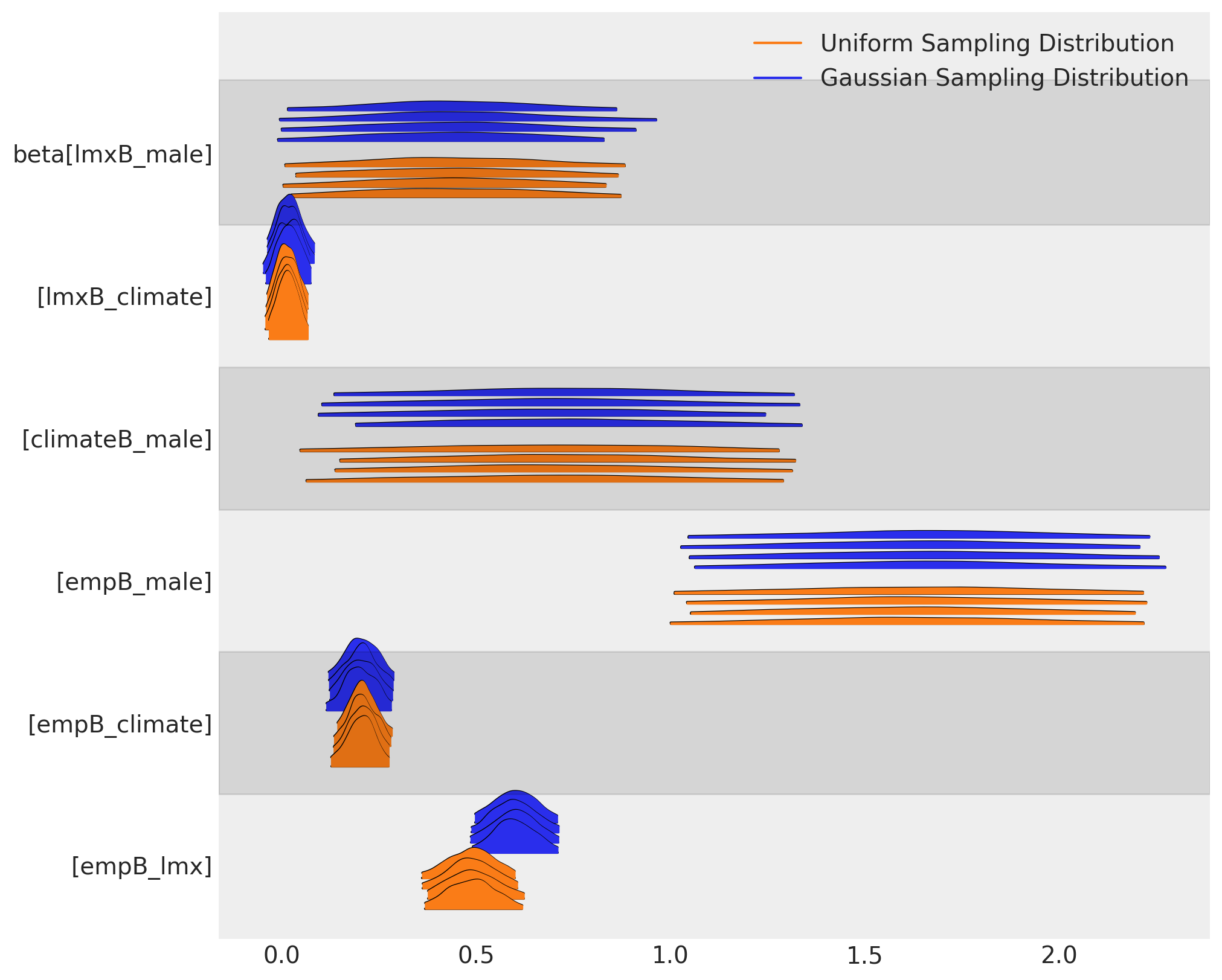This difference has downstream effects on the posterior predictive distribution. We can see here how the sampling distribution for the predictor terms influences the posterior predictive fits on our focal regression equation.

### Posterior Predictive Distributions#

az.plot_ppc(idata_uniform)

array([[<AxesSubplot: xlabel='climate_pred_observed / climate_pred_observed'>,
<AxesSubplot: xlabel='lmx_pred_observed / lmx_pred_observed'>,
<AxesSubplot: xlabel='lmx_imputed_observed / lmx_imputed_observed'>],
[<AxesSubplot: xlabel='climate_imputed_observed / climate_imputed_observed'>,
<AxesSubplot: xlabel='emp_imputed_observed / emp_imputed_observed'>,
<AxesSubplot: >]], dtype=object)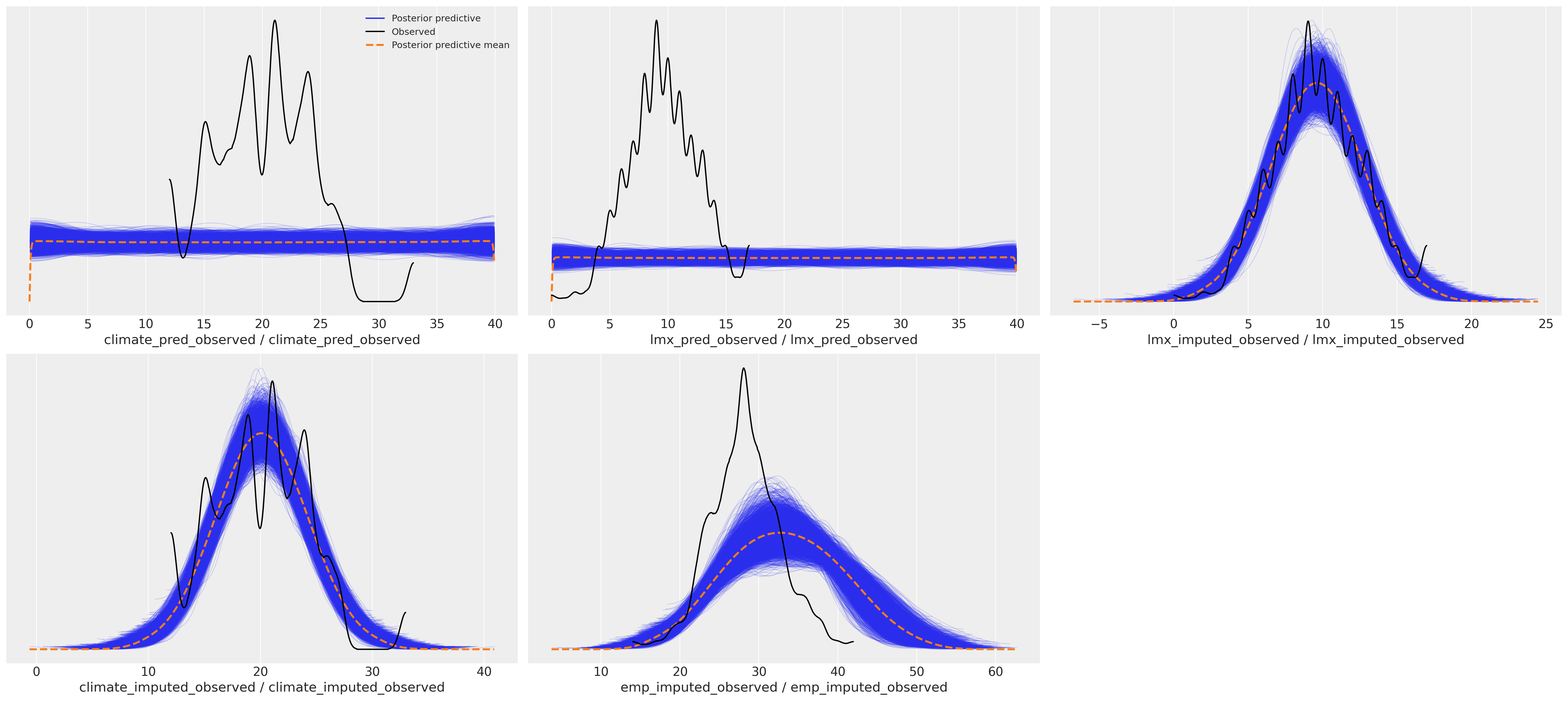az.plot_ppc(idata_normal)

array([[<AxesSubplot: xlabel='climate_pred_observed / climate_pred_observed'>,
<AxesSubplot: xlabel='lmx_pred_observed / lmx_pred_observed'>,
<AxesSubplot: xlabel='lmx_imputed_observed / lmx_imputed_observed'>],
[<AxesSubplot: xlabel='climate_imputed_observed / climate_imputed_observed'>,
<AxesSubplot: xlabel='emp_imputed_observed / emp_imputed_observed'>,
<AxesSubplot: >]], dtype=object)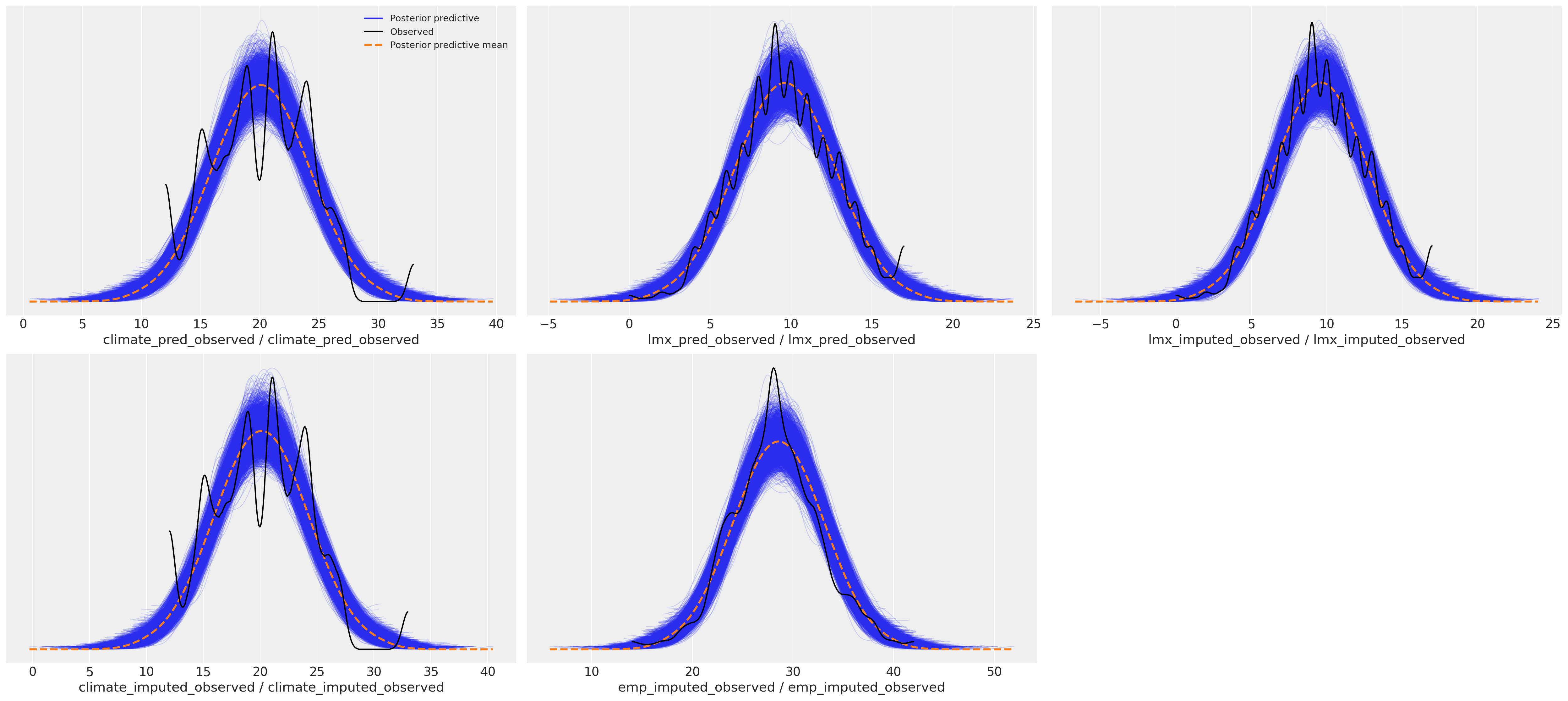### Process the Posterior Predictive Distribution#

Above we estimated a number of likelihood terms in a single PyMC model context. These likelihoods constrained the hyper-parameters which determined the imputation values of the missing terms in the variables used as predictors in our focal regression equation for empower. But we could also perform a more manual sequential imputation, where we model each of the subordinate regression equations seperately and extract the imputed values for each variable in turn and then run a simple regression on the imputed values for the focal regression equation.

We show here how to extract the imputed values for each of the regression equations and augment the observed data.

def get_imputed(idata, data):
imputed_data = data.copy()
imputed_climate = az.extract(idata, group="posterior_predictive", num_samples=1000)[
"climate_imputed"
].mean(axis=1)

imputed_lmx = az.extract(idata, group="posterior_predictive", num_samples=1000)[
"lmx_imputed"
].mean(axis=1)

imputed_emp = az.extract(idata, group="posterior_predictive", num_samples=1000)[
"emp_imputed"
].mean(axis=1)
assert imputed_data.isnull().sum().to_list() == [0, 0, 0, 0]
imputed_data.columns = ["imputed_" + col for col in imputed_data.columns]
return imputed_data

imputed_data_uniform = get_imputed(idata_uniform, data)
imputed_data_normal = get_imputed(idata_normal, data)

imputed_lmx imputed_empower imputed_climate imputed_male
0 11.0 32.000000 18.0 1
1 13.0 29.490539 18.0 1
2 9.0 30.000000 18.0 1
3 8.0 29.000000 18.0 1
4 7.0 26.000000 18.0 0

We used the mean here to impute the expected value for each missing cell, but you could perform a kind of sensitivity analysis using the many plausible values in posterior predictive distribution

### Plotting the Imputed Datasets#

Now we’ll plot the imputed values against their observed values to show how the different sampling distributions impact the pattern of imputation.

joined_uniform = pd.concat([imputed_data_uniform, data], axis=1)
joined_normal = pd.concat([imputed_data_normal, data], axis=1)
for col in ["lmx", "empower", "climate"]:
joined_uniform[col + "_missing"] = np.where(joined_uniform[col].isnull(), 1, 0)
joined_normal[col + "_missing"] = np.where(joined_normal[col].isnull(), 1, 0)

def rand_jitter(arr):
stdev = 0.01 * (max(arr) - min(arr))
return arr + np.random.randn(len(arr)) * stdev

fig, axs = plt.subplots(1, 3, figsize=(20, 8))
axs = axs.flatten()
ax = axs
ax1 = axs
ax2 = axs

## Derived from MV norm fit.
z = multivariate_normal(
[lmx_mean, joined_uniform["imputed_empower"].mean()], [[8.9, 5.4], [5.4, 19]]
).pdf(joined_uniform[["imputed_lmx", "imputed_empower"]])

ax.scatter(
rand_jitter(joined_uniform["imputed_lmx"]),
rand_jitter(joined_uniform["imputed_empower"]),
c=joined_uniform["empower_missing"],
cmap=cm.winter,
ec="black",
s=50,
)
ax.set_title("Relationship between LMX and Empowerment \n after Uniform Imputation", fontsize=20)
ax.tricontour(joined_uniform["imputed_lmx"], joined_uniform["imputed_empower"], z)
ax.set_ylabel("Empowerment")

custom_lines = [
Line2D(, , color=cm.winter(0.0), lw=4),
Line2D(, , color=cm.winter(0.9), lw=4),
]
ax.legend(custom_lines, ["Observed", "Missing - Imputed Empowerment Values"])

z = multivariate_normal(
[lmx_mean, joined_normal["imputed_empower"].mean()], [[8.9, 5.4], [5.4, 19]]
).pdf(joined_normal[["imputed_lmx", "imputed_empower"]])

ax2.scatter(
rand_jitter(joined_normal["imputed_lmx"]),
rand_jitter(joined_normal["imputed_empower"]),
c=joined_normal["empower_missing"],
cmap=cm.autumn,
ec="black",
s=50,
)
ax2.set_title("Relationship between LMX and Empowerment \n after Gaussian Imputation", fontsize=20)
ax2.tricontour(joined_normal["imputed_lmx"], joined_normal["imputed_empower"], z)
ax2.set_ylabel("Empowerment")
custom_lines = [
Line2D(, , color=cm.autumn(0.0), lw=4),
Line2D(, , color=cm.autumn(0.9), lw=4),
]
ax2.legend(custom_lines, ["Observed", "Missing - Imputed Empowerment Values"])

ax1.hist(
joined_normal["imputed_empower"],
label="Gaussian Imputed Empowerment",
bins=30,
color="slateblue",
ec="black",
)
ax1.hist(
joined_uniform["imputed_empower"],
label="Uniform Imputed Empowerment",
bins=30,
color="cyan",
ec="black",
)
ax1.hist(
joined_normal["empower"], label="Observed Empowerment", bins=30, color="magenta", ec="black"
)
ax1.legend()
ax1.set_title("Imputed & Observed Empowerment", fontsize=20);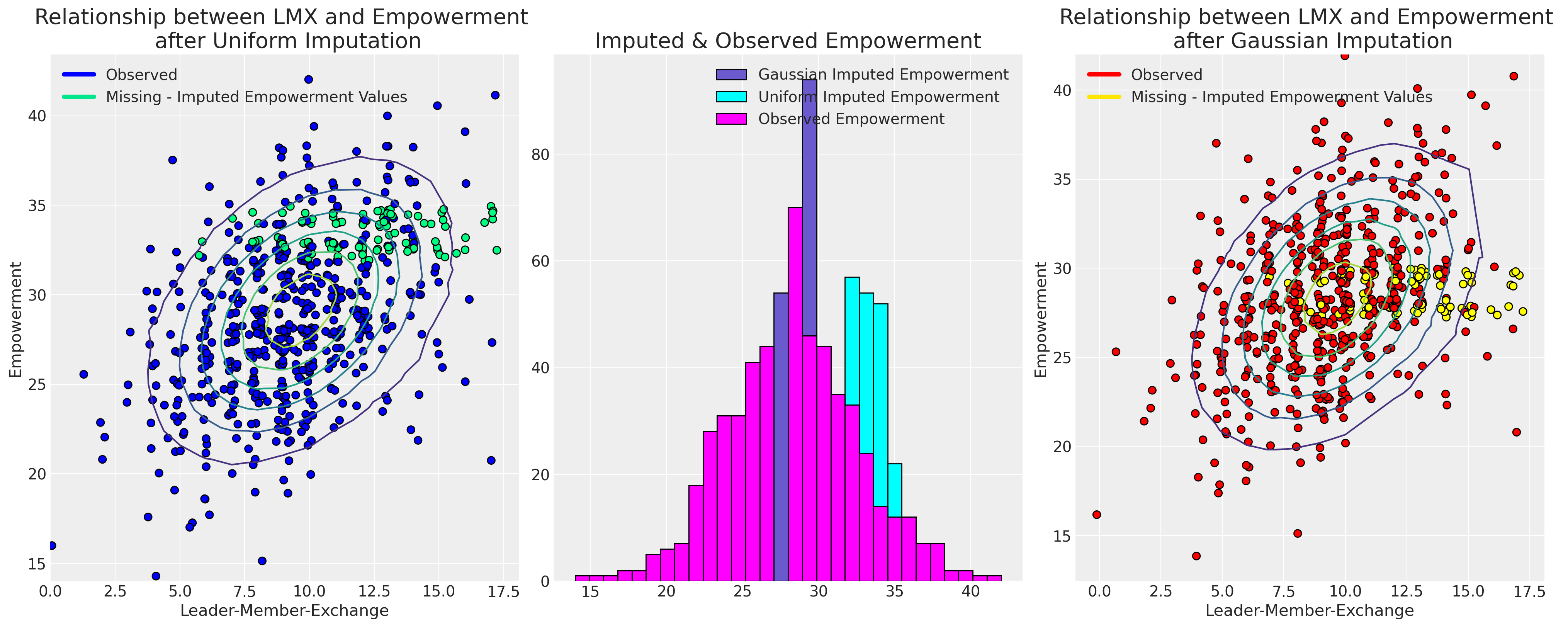Ultimately our choice of sampling distribution leads to differently plausible imputations. The choice of which model to go with will driven by the assumptions which govern the reasons for missing-ness in our data.

## Hierarchical Structures and Data Imputation#

Our employee dataset has more fine-grained structure than we’ve examined so far. In particular there are 100 or so teams which make up our employee pool, and we might wonder to what degree the propensity for satisfaction or incomplete survey scores are due to the local team environments? Could this be a factor in our patterns of missing data? We’ll examine the reported empowerment scores by team and plot the regression lines by as predicted within each team by their reported lmx score.

heatmap = df_employee.pivot("employee", "team", "empower").dropna(how="all")
heatmap = pd.concat(
[heatmap[~heatmap[col].isnull()][col].reset_index(drop=True) for col in heatmap.columns], axis=1
)
with pd.option_context("format.precision", 2):

/var/folders/99/gp2xl6x513s0tvl3cx79zf7m0000gn/T/ipykernel_96943/1805800404.py:1: FutureWarning: In a future version of pandas all arguments of DataFrame.pivot will be keyword-only.
heatmap = df_employee.pivot("employee", "team", "empower").dropna(how="all")

1 2 3 4 5 6 7 8 9 10 11 12 13 14 15 16 17 18 19 20 21 22 23 24 25 26 27 28 29 30 31 32 33 34 35 36 37 38 39 40 41 42 43 44 45 46 47 48 49 50 51 52 53 54 55 56 57 58 59 60 61 62 63 64 65 66 67 68 69 70 71 72 73 74 75 76 77 78 79 80 81 82 83 84 85 86 87 88 89 90 91 92 93 94 95 96 97 98 99 100 101 102 103 104 105
0 32.00 22.00 16.00 26.00 33.00 21.00 29.00 26.00 27.00 33.00 28.00 36.00 24.00 24.00 34.00 28.00 29.00 22.00 28.00 23.00 25.00 39.00 28.00 28.00 26.00 29.00 34.00 25.00 30.00 26.00 28.00 23.00 32.00 27.00 38.00 22.00 36.00 30.00 30.00 30.00 30.00 28.00 27.00 28.00 25.00 21.00 37.00 24.00 31.00 27.00 28.00 32.00 27.00 30.00 28.00 26.00 29.00 20.00 30.00 27.00 32.00 22.00 32.00 31.00 26.00 29.00 24.00 23.00 33.00 29.00 35.00 25.00 33.00 23.00 32.00 27.00 31.00 28.00 27.00 28.00 25.00 31.00 28.00 31.00 28.00 32.00 24.00 29.00 28.00 30.00 33.00 23.00 28.00 21.00 25.00 39.00 25.00 31.00 30.00 24.00 29.00 25.00 20.00 28.00 28.00
1 30.00 23.00 25.00 27.00 37.00 29.00 26.00 25.00 28.00 27.00 26.00 32.00 23.00 30.00 24.00 24.00 26.00 28.00 33.00 22.00 17.00 31.00 22.00 36.00 34.00 23.00 32.00 30.00 30.00 22.00 22.00 28.00 31.00 30.00 32.00 23.00 32.00 36.00 23.00 26.00 24.00 32.00 36.00 26.00 25.00 35.00 32.00 28.00 24.00 28.00 35.00 28.00 32.00 24.00 26.00 23.00 26.00 29.00 28.00 28.00 33.00 29.00 25.00 28.00 27.00 29.00 24.00 34.00 27.00 28.00 31.00 27.00 25.00 30.00 28.00 20.00 28.00 32.00 23.00 15.00 29.00 31.00 31.00 28.00 30.00 28.00 40.00 30.00 26.00 19.00 25.00 23.00 32.00 27.00 30.00 26.00 35.00 24.00 25.00 23.00 28.00 34.00 26.00 28.00 17.00
2 29.00 32.00 31.00 42.00 29.00 25.00 26.00 29.00 26.00 29.00 30.00 30.00 25.00 22.00 21.00 34.00 33.00 32.00 26.00 29.00 35.00 32.00 33.00 27.00 26.00 22.00 29.00 29.00 32.00 30.00 35.00 29.00 33.00 30.00 30.00 31.00 26.00 28.00 40.00 25.00 41.00 27.00 23.00 31.00 29.00 28.00 27.00 23.00 36.00 28.00 23.00 31.00 29.00 33.00 27.00 19.00 25.00 33.00 29.00 27.00 23.00 28.00 31.00 26.00 22.00 37.00 24.00 33.00 37.00 29.00 29.00 26.00 27.00 31.00 23.00 14.00 28.00 30.00 29.00 28.00 36.00 27.00 28.00 35.00 29.00 38.00 26.00 38.00 30.00 34.00 38.00 28.00 34.00 28.00 28.00 30.00 31.00 27.00 29.00 24.00 33.00 30.00 28.00 26.00 28.00
3 26.00 36.00 27.00 24.00 32.00 36.00 26.00 27.00 29.00 36.00 28.00 30.00 27.00 27.00 33.00 34.00 29.00 27.00 33.00 26.00 26.00 33.00 30.00 26.00 28.00 31.00 20.00 30.00 23.00 30.00 28.00 25.00 32.00 31.00 18.00 29.00 26.00 26.00 27.00 nan 28.00 nan 29.00 25.00 22.00 33.00 33.00 30.00 33.00 34.00 nan 37.00 29.00 27.00 28.00 23.00 25.00 32.00 21.00 24.00 30.00 29.00 28.00 27.00 24.00 38.00 24.00 19.00 30.00 35.00 32.00 28.00 38.00 31.00 27.00 23.00 30.00 27.00 27.00 27.00 32.00 27.00 29.00 26.00 24.00 29.00 28.00 31.00 25.00 25.00 30.00 29.00 34.00 32.00 31.00 26.00 nan 34.00 27.00 21.00 24.00 25.00 28.00 23.00 32.00
4 nan nan 30.00 37.00 24.00 nan 31.00 nan 28.00 24.00 28.00 34.00 24.00 38.00 35.00 nan nan nan nan 29.00 37.00 32.00 nan 24.00 nan 26.00 29.00 26.00 35.00 29.00 nan 29.00 nan nan nan 20.00 23.00 31.00 22.00 nan nan nan 23.00 nan 19.00 nan 32.00 22.00 31.00 27.00 nan nan nan nan 24.00 nan 27.00 28.00 26.00 25.00 30.00 22.00 30.00 28.00 32.00 29.00 28.00 nan nan 28.00 30.00 nan 28.00 26.00 25.00 nan 27.00 35.00 24.00 29.00 24.00 nan 33.00 28.00 34.00 31.00 22.00 nan 26.00 18.00 32.00 22.00 nan 31.00 33.00 nan nan 32.00 28.00 21.00 35.00 36.00 31.00 27.00 nan
5 nan nan 23.00 nan 31.00 nan 33.00 nan 25.00 22.00 25.00 nan nan 30.00 23.00 nan nan nan nan 24.00 nan 31.00 nan nan nan nan nan nan nan 32.00 nan 25.00 nan nan nan 20.00 31.00 25.00 nan nan nan nan nan nan 28.00 nan nan 27.00 27.00 nan nan nan nan nan 27.00 nan 31.00 29.00 nan 31.00 nan 30.00 nan nan nan nan nan nan nan nan 28.00 nan nan nan nan nan 33.00 30.00 19.00 23.00 nan nan 26.00 28.00 26.00 nan nan nan 28.00 30.00 36.00 24.00 nan nan 29.00 nan nan nan 28.00 27.00 28.00 31.00 24.00 nan nan
fits = []
x = np.linspace(0, 20, 100)
fig, ax = plt.subplots(figsize=(20, 7))
for team in df_employee["team"].unique():
temp = df_employee[df_employee["team"] == team][["lmx", "empower"]].dropna()
fit = np.polyfit(temp["lmx"], temp["empower"], 1)
y = fit * x + fit
fits.append(fit)
ax.plot(x, y, alpha=0.6)
ax.scatter(rand_jitter(temp["lmx"]), rand_jitter(temp["empower"]), color="black", ec="white")
ax.set_title("Simple Regression fits by Team \n Empower ~ LMX", fontsize=20)
ax.set_ylabel("Empowerment")
ax.set_ylim(0, 45);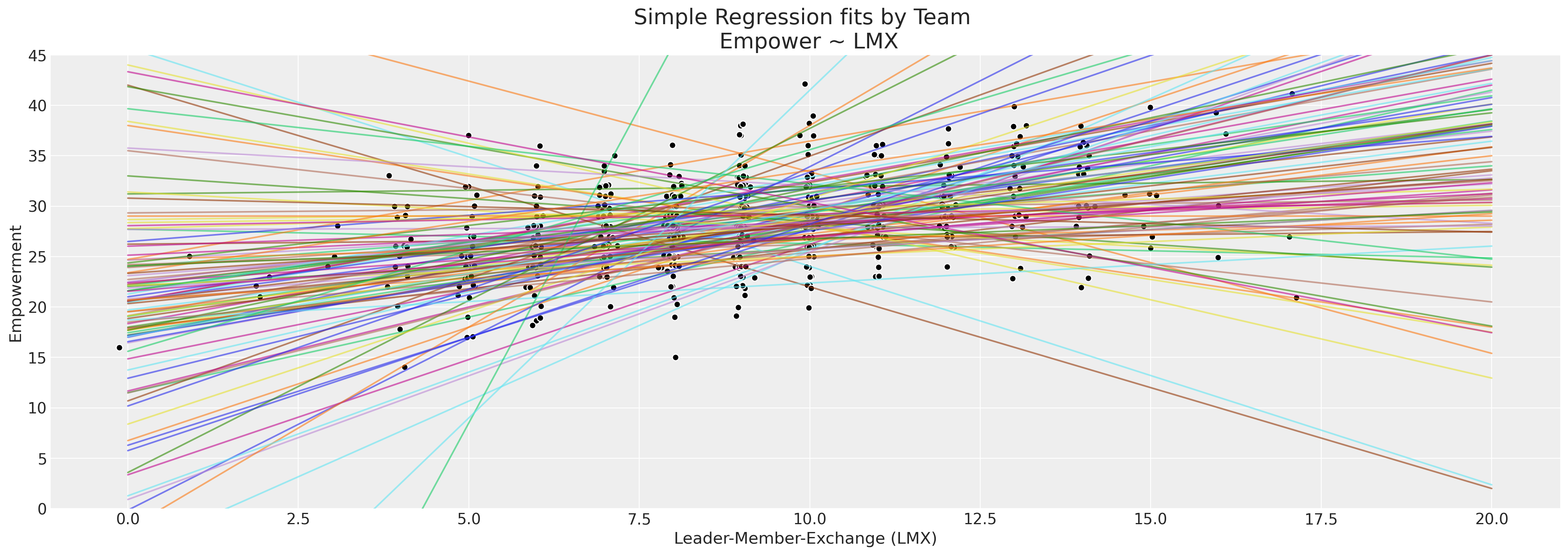There is enough spread in the regression lines to at least suggest that there is a heterogenous relationship between empowerment and the work environment as we look across different teams, but limited observations in each team. This is a perfect use case for a hierarchical bayesian model.

team_idx, teams = pd.factorize(df_employee["team"], sort=True)
employee_idx, _ = pd.factorize(df_employee["employee"], sort=True)
coords = {"team": teams, "employee": np.arange(len(df_employee))}

with pm.Model(coords=coords) as hierarchical_model:
# Priors
company_beta_lmx = pm.Normal("company_beta_lmx", 0, 1)
company_beta_male = pm.Normal("company_beta_male", 0, 1)
company_alpha = pm.Normal("company_alpha", 20, 2)
team_alpha = pm.Normal("team_alpha", 0, 1, dims="team")
team_beta_lmx = pm.Normal("team_beta_lmx", 0, 1, dims="team")
sigma = pm.HalfNormal("sigma", 4, dims="employee")

# Imputed Predictors
mu_lmx = pm.Normal("mu_lmx", 10, 5)
sigma_lmx = pm.HalfNormal("sigma_lmx", 5)
lmx_pred = pm.Normal("lmx_pred", mu_lmx, sigma_lmx, observed=df_employee["lmx"].values)

# Combining Levels
alpha_global = pm.Deterministic("alpha_global", company_alpha + team_alpha[team_idx])
beta_global_lmx = pm.Deterministic(
"beta_global_lmx", company_beta_lmx + team_beta_lmx[team_idx]
)
beta_global_male = pm.Deterministic("beta_global_male", company_beta_male)

# Likelihood
mu = pm.Deterministic(
"mu",
alpha_global + beta_global_lmx * lmx_pred + beta_global_male * df_employee["male"].values,
)

empower_imputed = pm.Normal(
"emp_imputed",
mu,
sigma,
observed=df_employee["empower"].values,
)

idata_hierarchical = pm.sample_prior_predictive()
# idata_hierarchical.extend(pm.sample(random_seed=1200, target_accept=0.99))
idata_hierarchical.extend(
sample_blackjax_nuts(draws=20_000, random_seed=500, target_accept=0.99)
)
pm.sample_posterior_predictive(idata_hierarchical, extend_inferencedata=True)

pm.model_to_graphviz(hierarchical_model)

/Users/nathanielforde/opt/miniconda3/envs/missing_data_clean/lib/python3.11/site-packages/pymc/model.py:1400: ImputationWarning: Data in lmx_pred contains missing values and will be automatically imputed from the sampling distribution.
warnings.warn(impute_message, ImputationWarning)
/Users/nathanielforde/opt/miniconda3/envs/missing_data_clean/lib/python3.11/site-packages/pymc/model.py:1400: ImputationWarning: Data in emp_imputed contains missing values and will be automatically imputed from the sampling distribution.
warnings.warn(impute_message, ImputationWarning)
Sampling: [company_alpha, company_beta_lmx, company_beta_male, emp_imputed_missing, emp_imputed_observed, lmx_pred_missing, lmx_pred_observed, mu_lmx, sigma, sigma_lmx, team_alpha, team_beta_lmx]

Compiling...
Compilation time =  0:00:04.523249
Sampling...
Sampling time =  0:00:12.370856
Transforming variables...
Transformation time =  0:12:51.685820

Sampling: [emp_imputed_missing, emp_imputed_observed, lmx_pred_observed]

100.00% [80000/80000 00:21<00:00]idata_hierarchical

arviz.InferenceData
• <xarray.Dataset>
Dimensions:                    (chain: 4, draw: 20000, team: 105,
lmx_pred_missing_dim_0: 26,
emp_imputed_missing_dim_0: 102, employee: 630,
lmx_pred_dim_0: 630, alpha_global_dim_0: 630,
beta_global_lmx_dim_0: 630, mu_dim_0: 630,
emp_imputed_dim_0: 630)
Coordinates:
* chain                      (chain) int64 0 1 2 3
* draw                       (draw) int64 0 1 2 3 ... 19996 19997 19998 19999
* team                       (team) int64 1 2 3 4 5 6 ... 101 102 103 104 105
* lmx_pred_missing_dim_0     (lmx_pred_missing_dim_0) int64 0 1 2 ... 23 24 25
* emp_imputed_missing_dim_0  (emp_imputed_missing_dim_0) int64 0 1 ... 100 101
* employee                   (employee) int64 0 1 2 3 4 ... 626 627 628 629
* lmx_pred_dim_0             (lmx_pred_dim_0) int64 0 1 2 3 ... 627 628 629
* alpha_global_dim_0         (alpha_global_dim_0) int64 0 1 2 ... 627 628 629
* beta_global_lmx_dim_0      (beta_global_lmx_dim_0) int64 0 1 2 ... 628 629
* mu_dim_0                   (mu_dim_0) int64 0 1 2 3 4 ... 626 627 628 629
* emp_imputed_dim_0          (emp_imputed_dim_0) int64 0 1 2 3 ... 627 628 629
Data variables: (12/16)
company_beta_lmx           (chain, draw) float64 0.6299 0.6698 ... 0.7356
company_beta_male          (chain, draw) float64 0.8914 0.9321 ... 0.9751
company_alpha              (chain, draw) float64 21.29 21.02 ... 20.83 20.77
team_alpha                 (chain, draw, team) float64 -1.535 ... 0.1378
team_beta_lmx              (chain, draw, team) float64 0.3924 ... -0.1927
mu_lmx                     (chain, draw) float64 9.773 9.815 ... 9.797 9.764
...                         ...
lmx_pred                   (chain, draw, lmx_pred_dim_0) float64 11.0 ......
alpha_global               (chain, draw, alpha_global_dim_0) float64 19.7...
beta_global_lmx            (chain, draw, beta_global_lmx_dim_0) float64 1...
beta_global_male           (chain, draw) float64 0.8914 0.9321 ... 0.9751
mu                         (chain, draw, mu_dim_0) float64 31.89 ... 24.59
emp_imputed                (chain, draw, emp_imputed_dim_0) float64 32.0 ...
Attributes:
created_at:     2023-02-02T08:13:38.333014
arviz_version:  0.14.0

• <xarray.Dataset>
Dimensions:                     (chain: 4, draw: 20000,
lmx_pred_observed_dim_2: 604,
emp_imputed_observed_dim_2: 528,
lmx_pred_dim_2: 630, mu_dim_2: 630,
emp_imputed_dim_2: 630)
Coordinates:
* chain                       (chain) int64 0 1 2 3
* draw                        (draw) int64 0 1 2 3 ... 19996 19997 19998 19999
* lmx_pred_observed_dim_2     (lmx_pred_observed_dim_2) int64 0 1 ... 602 603
* emp_imputed_observed_dim_2  (emp_imputed_observed_dim_2) int64 0 1 ... 527
* lmx_pred_dim_2              (lmx_pred_dim_2) int64 0 1 2 3 ... 627 628 629
* mu_dim_2                    (mu_dim_2) int64 0 1 2 3 4 ... 626 627 628 629
* emp_imputed_dim_2           (emp_imputed_dim_2) int64 0 1 2 ... 627 628 629
Data variables:
lmx_pred_observed           (chain, draw, lmx_pred_observed_dim_2) float64 ...
emp_imputed_observed        (chain, draw, emp_imputed_observed_dim_2) float64 ...
lmx_pred                    (chain, draw, lmx_pred_dim_2) float64 14.09 ....
mu                          (chain, draw, mu_dim_2) float64 35.05 ... 24.5
emp_imputed                 (chain, draw, emp_imputed_dim_2) float64 34.7...
Attributes:
created_at:                 2023-02-02T08:14:02.072909
arviz_version:              0.14.0
inference_library:          pymc
inference_library_version:  5.0.1

• <xarray.Dataset>
Dimensions:          (chain: 4, draw: 20000)
Coordinates:
* chain            (chain) int64 0 1 2 3
* draw             (draw) int64 0 1 2 3 4 5 ... 19995 19996 19997 19998 19999
Data variables:
lp               (chain, draw) float64 4.1e+03 4.134e+03 ... 4.072e+03
diverging        (chain, draw) bool False False False ... False False False
energy           (chain, draw) float64 4.569e+03 4.597e+03 ... 4.562e+03
tree_depth       (chain, draw) int64 10 10 10 10 10 10 ... 10 10 10 10 10 10
n_steps          (chain, draw) int64 1023 1023 1023 1023 ... 1023 1023 1023
acceptance_rate  (chain, draw) float64 0.9823 0.9843 ... 0.9916 0.9895
Attributes:
created_at:     2023-02-02T08:13:38.402578
arviz_version:  0.14.0

• <xarray.Dataset>
Dimensions:                    (chain: 1, draw: 500,
lmx_pred_missing_dim_0: 26,
lmx_pred_dim_0: 630, team: 105,
alpha_global_dim_0: 630,
beta_global_lmx_dim_0: 630,
emp_imputed_missing_dim_0: 102, mu_dim_0: 630,
employee: 630, emp_imputed_dim_0: 630)
Coordinates:
* chain                      (chain) int64 0
* draw                       (draw) int64 0 1 2 3 4 5 ... 495 496 497 498 499
* lmx_pred_missing_dim_0     (lmx_pred_missing_dim_0) int64 0 1 2 ... 23 24 25
* lmx_pred_dim_0             (lmx_pred_dim_0) int64 0 1 2 3 ... 627 628 629
* team                       (team) int64 1 2 3 4 5 6 ... 101 102 103 104 105
* alpha_global_dim_0         (alpha_global_dim_0) int64 0 1 2 ... 627 628 629
* beta_global_lmx_dim_0      (beta_global_lmx_dim_0) int64 0 1 2 ... 628 629
* emp_imputed_missing_dim_0  (emp_imputed_missing_dim_0) int64 0 1 ... 100 101
* mu_dim_0                   (mu_dim_0) int64 0 1 2 3 4 ... 626 627 628 629
* employee                   (employee) int64 0 1 2 3 4 ... 626 627 628 629
* emp_imputed_dim_0          (emp_imputed_dim_0) int64 0 1 2 3 ... 627 628 629
Data variables: (12/16)
company_alpha              (chain, draw) float64 18.23 21.82 ... 23.99 18.59
beta_global_male           (chain, draw) float64 -1.439 -0.3283 ... -0.8552
lmx_pred_missing           (chain, draw, lmx_pred_missing_dim_0) float64 ...
company_beta_lmx           (chain, draw) float64 -0.008152 1.042 ... 0.29
lmx_pred                   (chain, draw, lmx_pred_dim_0) float64 13.11 .....
team_alpha                 (chain, draw, team) float64 1.207 ... 0.9462
...                         ...
emp_imputed_missing        (chain, draw, emp_imputed_missing_dim_0) float64 ...
mu                         (chain, draw, mu_dim_0) float64 43.46 ... 25.64
team_beta_lmx              (chain, draw, team) float64 1.951 ... 0.2287
sigma                      (chain, draw, employee) float64 5.371 ... 4.738
emp_imputed                (chain, draw, emp_imputed_dim_0) float64 35.27...
mu_lmx                     (chain, draw) float64 13.31 13.64 ... 9.915 9.307
Attributes:
created_at:                 2023-02-02T08:00:29.477993
arviz_version:              0.14.0
inference_library:          pymc
inference_library_version:  5.0.1

• <xarray.Dataset>
Dimensions:                     (chain: 1, draw: 500,
lmx_pred_observed_dim_0: 604,
emp_imputed_observed_dim_0: 528)
Coordinates:
* chain                       (chain) int64 0
* draw                        (draw) int64 0 1 2 3 4 5 ... 495 496 497 498 499
* lmx_pred_observed_dim_0     (lmx_pred_observed_dim_0) int64 0 1 ... 602 603
* emp_imputed_observed_dim_0  (emp_imputed_observed_dim_0) int64 0 1 ... 527
Data variables:
lmx_pred_observed           (chain, draw, lmx_pred_observed_dim_0) float64 ...
emp_imputed_observed        (chain, draw, emp_imputed_observed_dim_0) float64 ...
Attributes:
created_at:                 2023-02-02T08:00:29.484585
arviz_version:              0.14.0
inference_library:          pymc
inference_library_version:  5.0.1

• <xarray.Dataset>
Dimensions:                     (lmx_pred_observed_dim_0: 604,
emp_imputed_observed_dim_0: 528)
Coordinates:
* lmx_pred_observed_dim_0     (lmx_pred_observed_dim_0) int64 0 1 ... 602 603
* emp_imputed_observed_dim_0  (emp_imputed_observed_dim_0) int64 0 1 ... 527
Data variables:
lmx_pred_observed           (lmx_pred_observed_dim_0) float64 11.0 ... 5.0
emp_imputed_observed        (emp_imputed_observed_dim_0) float64 32.0 ......
Attributes:
created_at:                 2023-02-02T08:00:29.485965
arviz_version:              0.14.0
inference_library:          pymc
inference_library_version:  5.0.1

### Some Convergence Checks#

az.plot_trace(
idata_hierarchical,
var_names=["company_alpha", "team_alpha", "company_beta_lmx", "team_beta_lmx"],
kind="rank_vlines",
);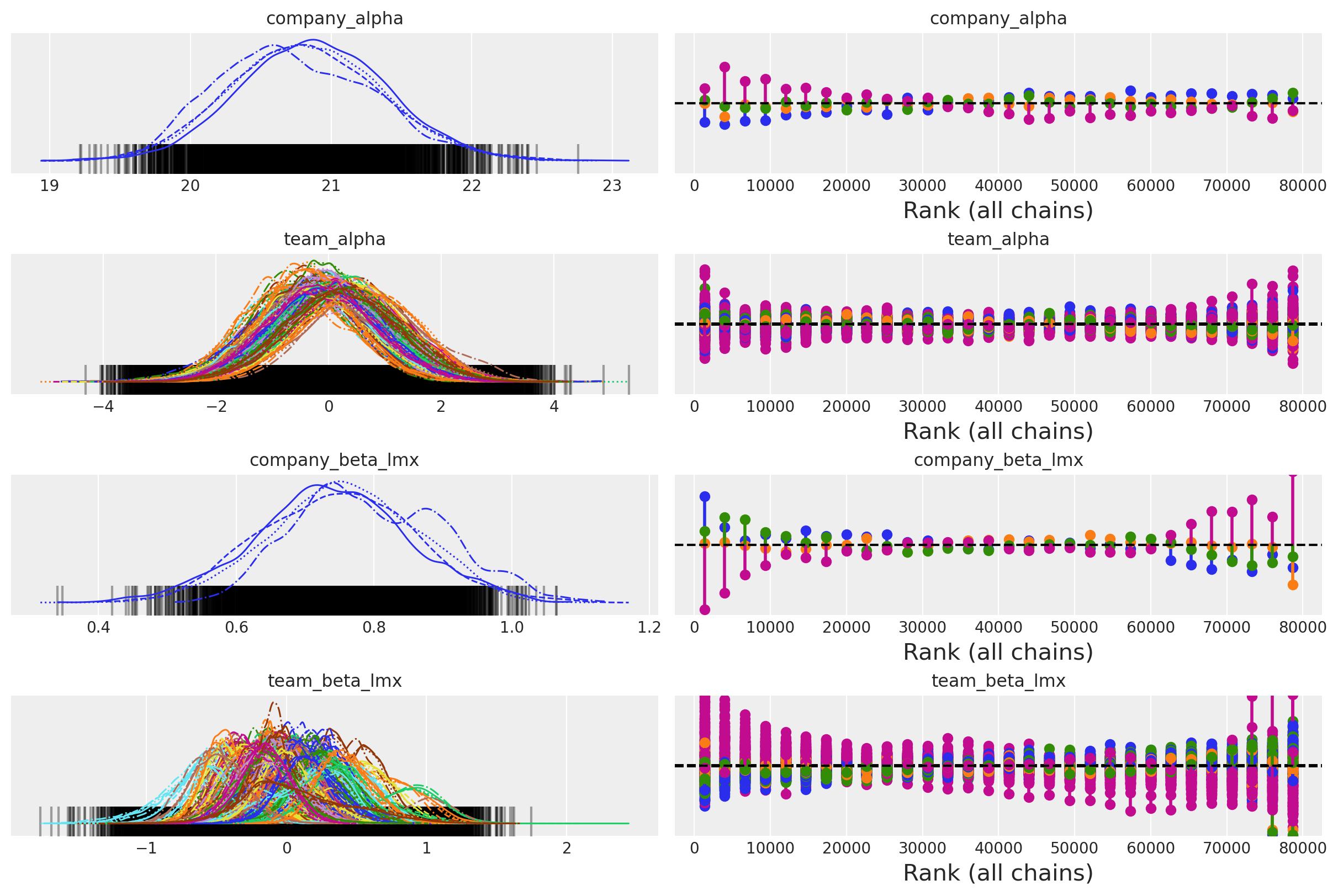az.plot_energy(idata_hierarchical, figsize=(20, 7));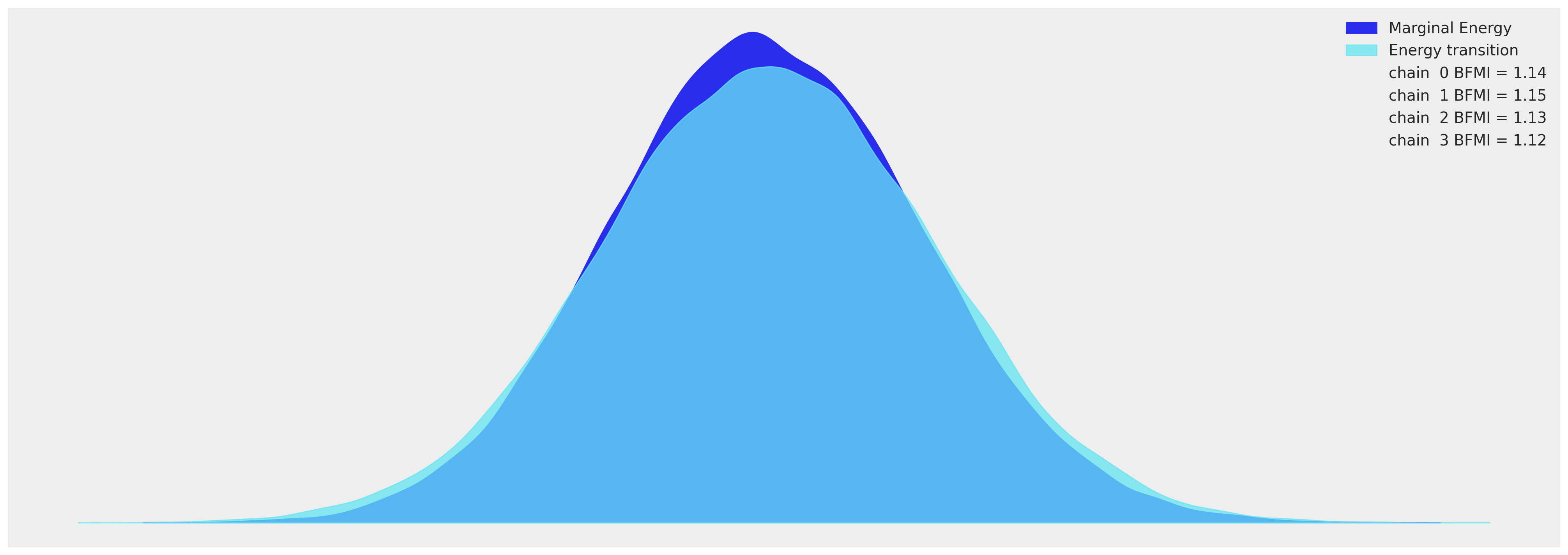### Inspecting the Model Fit#

summary = az.summary(
idata_hierarchical,
var_names=[
"company_alpha",
"team_alpha",
"company_beta_lmx",
"company_beta_male",
"team_beta_lmx",
],
)

summary

mean sd hdi_3% hdi_97% mcse_mean mcse_sd ess_bulk ess_tail r_hat
company_alpha 20.818 0.545 19.806 21.840 0.029 0.020 358.0 1316.0 1.02
team_alpha -0.214 0.955 -1.975 1.604 0.030 0.021 1043.0 2031.0 1.00
team_alpha -0.067 0.995 -1.975 1.772 0.026 0.018 1496.0 2572.0 1.00
team_alpha -0.568 0.931 -2.271 1.250 0.027 0.019 1144.0 2135.0 1.00
team_alpha -0.228 0.993 -2.085 1.630 0.025 0.018 1552.0 4305.0 1.00
... ... ... ... ... ... ... ... ... ...
team_beta_lmx 0.157 0.207 -0.226 0.550 0.010 0.007 436.0 872.0 1.01
team_beta_lmx 0.407 0.198 0.042 0.785 0.011 0.008 338.0 876.0 1.01
team_beta_lmx -0.146 0.213 -0.549 0.253 0.014 0.010 215.0 835.0 1.03
team_beta_lmx -0.167 0.187 -0.517 0.186 0.010 0.007 338.0 1346.0 1.01
team_beta_lmx 0.071 0.393 -0.562 0.902 0.021 0.015 390.0 476.0 1.01

213 rows × 9 columns

az.plot_ppc(
idata_hierarchical, var_names=["emp_imputed_observed"], figsize=(20, 7), num_pp_samples=1000
)

<AxesSubplot: xlabel='emp_imputed_observed / emp_imputed_observed'>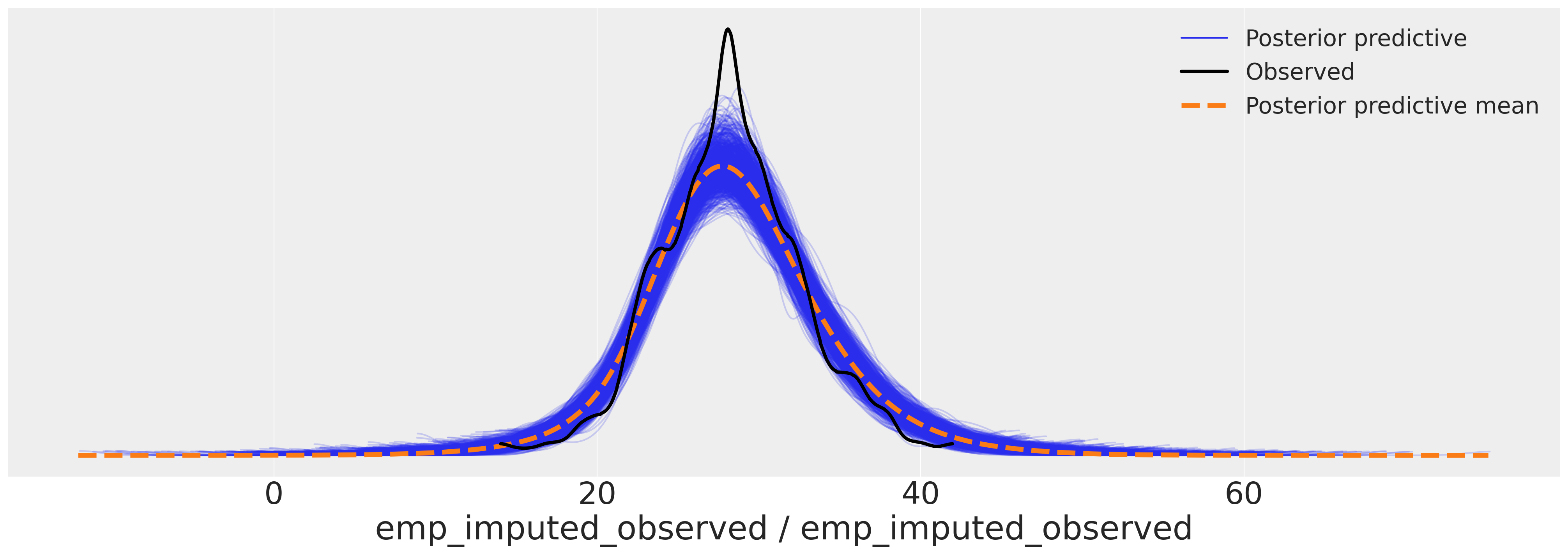### Heterogenous Patterns of Imputation#

Just as when we consider questions of causal inference and we attend to the confounding influence of local factors, this is also required when we do imputation. We show here a selection of team specific intercept terms which suggest that belonging to a particular team can shift your empowerment above or below the grand mean of the company level intercept term. These local effects of environment are what we seek to account for when imputing missing values.

ax = az.plot_forest(
idata_hierarchical,
var_names=["team_beta_lmx"],
coords={"team": [1, 20, 22, 30, 50, 70, 76, 80, 100]},
figsize=(20, 15),
kind="ridgeplot",
combined=True,
ridgeplot_alpha=0.4,
hdi_prob=True,
)
ax.axvline(0)
ax.set_title("Team Contribution to the marginal effect of LMX on Empowerment", fontsize=20);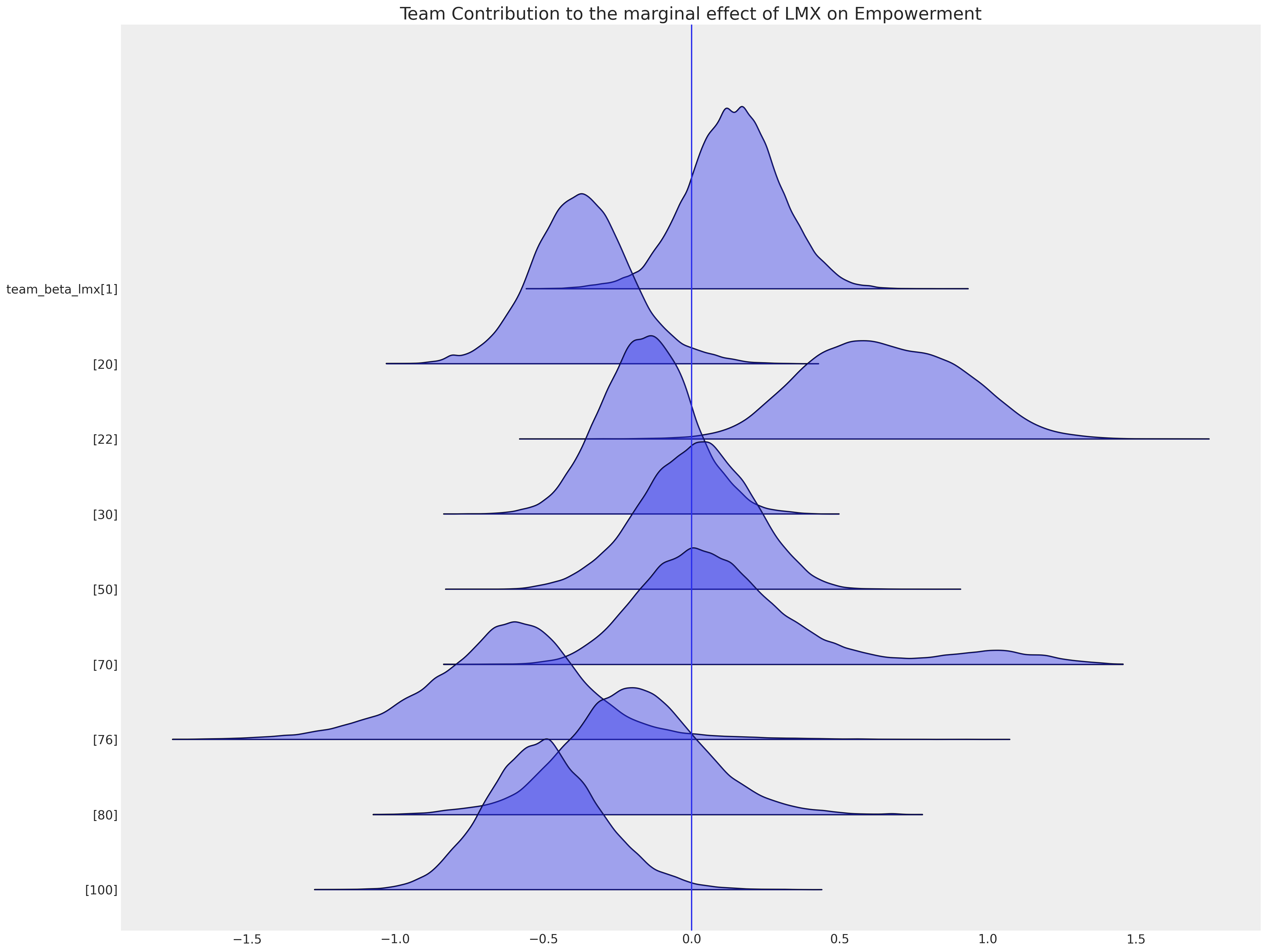The ability to capture this local variation impacts the pattern of imputed values too.

imputed_data = df_employee[["lmx", "empower", "climate"]]

imputed_lmx = az.extract(idata_hierarchical, group="posterior_predictive", num_samples=1000)[
"lmx_pred"
].mean(axis=1)

imputed_emp = az.extract(idata_hierarchical, group="posterior_predictive", num_samples=1000)[
"emp_imputed"
].mean(axis=1)
imputed_data.columns = ["imputed_" + col for col in imputed_data.columns]
joined = pd.concat([imputed_data, df_employee], axis=1)
joined["check"] = np.where(joined["empower"].isnull(), 1, 0)

mosaic = """AAAABB"""
fig, axs = plt.subplot_mosaic(mosaic, sharex=False, figsize=(20, 7))
axs = [axs[k] for k in axs.keys()]
axs.scatter(
joined["imputed_lmx"],
joined["imputed_empower"],
c=joined["check"],
cmap=cm.winter,
ec="black",
s=40,
)

z = multivariate_normal([10, joined["imputed_empower"].mean()], [[8.9, 5.4], [5.4, 19]]).pdf(
joined[["imputed_lmx", "imputed_empower"]]
)
axs.tricontour(joined["imputed_lmx"], joined["imputed_empower"], z)

axs.hist(joined["imputed_empower"], ec="black", label="Imputed", color="limegreen", bins=30)
axs.hist(joined["empower"], ec="black", label="observed", color="blue", bins=30)
axs.set_title("Empowerment Distributions Imputed  \n with Team Informed Estimates", fontsize=20)
axs.set_ylabel("Empowerment")
axs.set_title("Empowerment Imputed \n with Team Informed Estimates", fontsize=20)
axs.legend();

/var/folders/99/gp2xl6x513s0tvl3cx79zf7m0000gn/T/ipykernel_96943/3267370214.py:7: SettingWithCopyWarning:
A value is trying to be set on a copy of a slice from a DataFrame

See the caveats in the documentation: https://pandas.pydata.org/pandas-docs/stable/user_guide/indexing.html#returning-a-view-versus-a-copy
/var/folders/99/gp2xl6x513s0tvl3cx79zf7m0000gn/T/ipykernel_96943/3267370214.py:13: SettingWithCopyWarning:
A value is trying to be set on a copy of a slice from a DataFrame

See the caveats in the documentation: https://pandas.pydata.org/pandas-docs/stable/user_guide/indexing.html#returning-a-view-versus-a-copy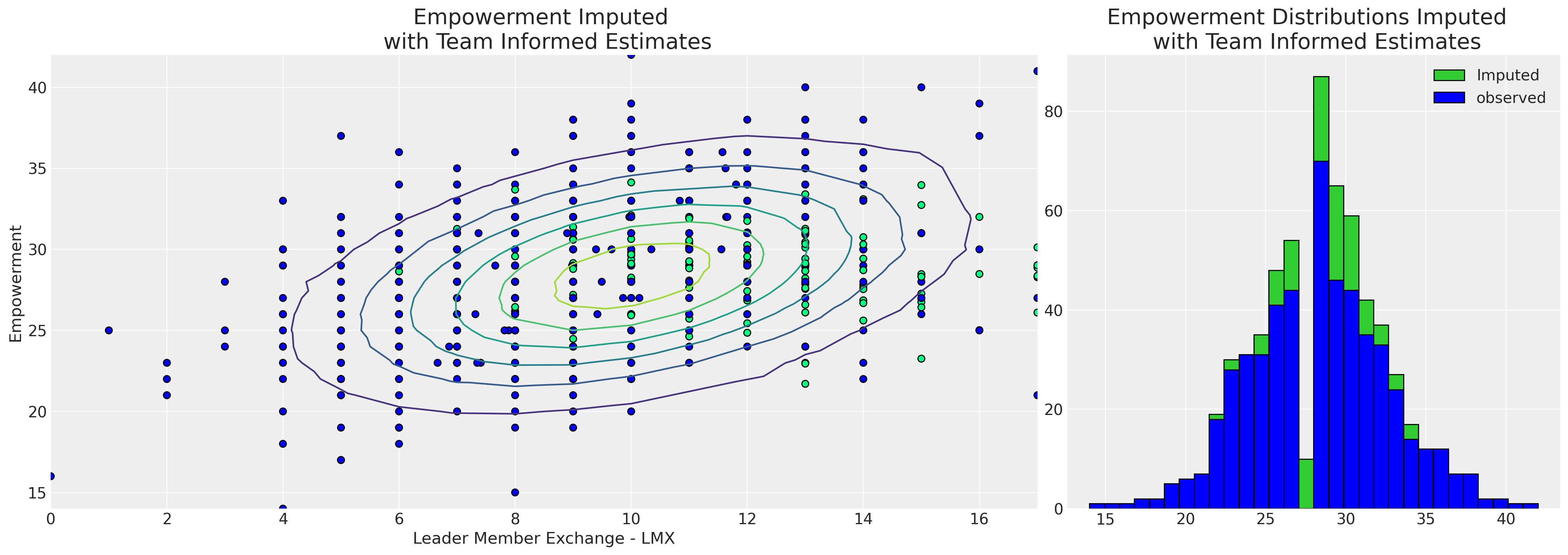It’s clear from the hierarchical model that the team specific information has allowed us to impute a wider range of empowerment values with a broader spread as a function of lmx and male. This is much more persuasive since all politics is local, and this latter model is informed by the conditions of work for each employee. As such, our hierarchical model is able to ascribe a more nuanced view of the probable empowerment values for the missing reports. The hierarchical imputation model “borrows information” in two ways (i) the individual team estimates are pulled toward the global estimates and (ii) the missing values are imputed with respect to our measures of the team dynamics.

## Conclusion#

We’ve now seen multiple approaches to the imputation of missing data. We have focused on an example where the reason for the missing data is not immediately obvious given how different employees might very well have different reasons for under-specifying their relationship with management. However the techniques applied here are quite general.

The multivariate normal approaches to imputation works surprisingly well in many cases, but the more cutting edge approach is the sequential specification of chained equations. The Bayesian approach here is state of the art because we are quite free to use more than simple regression models as the component models for our imputation equations. For each equation we can be liberal in our choice of likelihood terms and the priors we allow over the sampling distributions. We can also add hierarchical structure to respect natural clusters in our data in so far as they constrain the patterns of missing data.

This general point is important - the flexibility of the Bayesian approach can be tailored to the appropriate complexity of our theory about why our data is missing. Similar considerations apply to the estimation procedures involved in counterfactual inference. The more developed our theory for why the data is missing (why the world is as it is, and not another way), the more we need a flexible modelling framework to capture the subtleties of the theory. Bayesian modelling is a superb tool for this loop of theory construction and evaluation.

## Watermark#

%load_ext watermark
%watermark -n -u -v -iv -w -p pytensor

Last updated: Thu Feb 02 2023

Python implementation: CPython
Python version       : 3.11.0
IPython version      : 8.8.0

pytensor: 2.8.11

sys       : 3.11.0 | packaged by conda-forge | (main, Jan 15 2023, 05:44:48) [Clang 14.0.6 ]
pytensor  : 2.8.11
scipy     : 1.10.0
pymc      : 5.0.1
numpy     : 1.24.1
matplotlib: 3.6.3
arviz     : 0.14.0
pandas    : 1.5.2

Watermark: 2.3.1